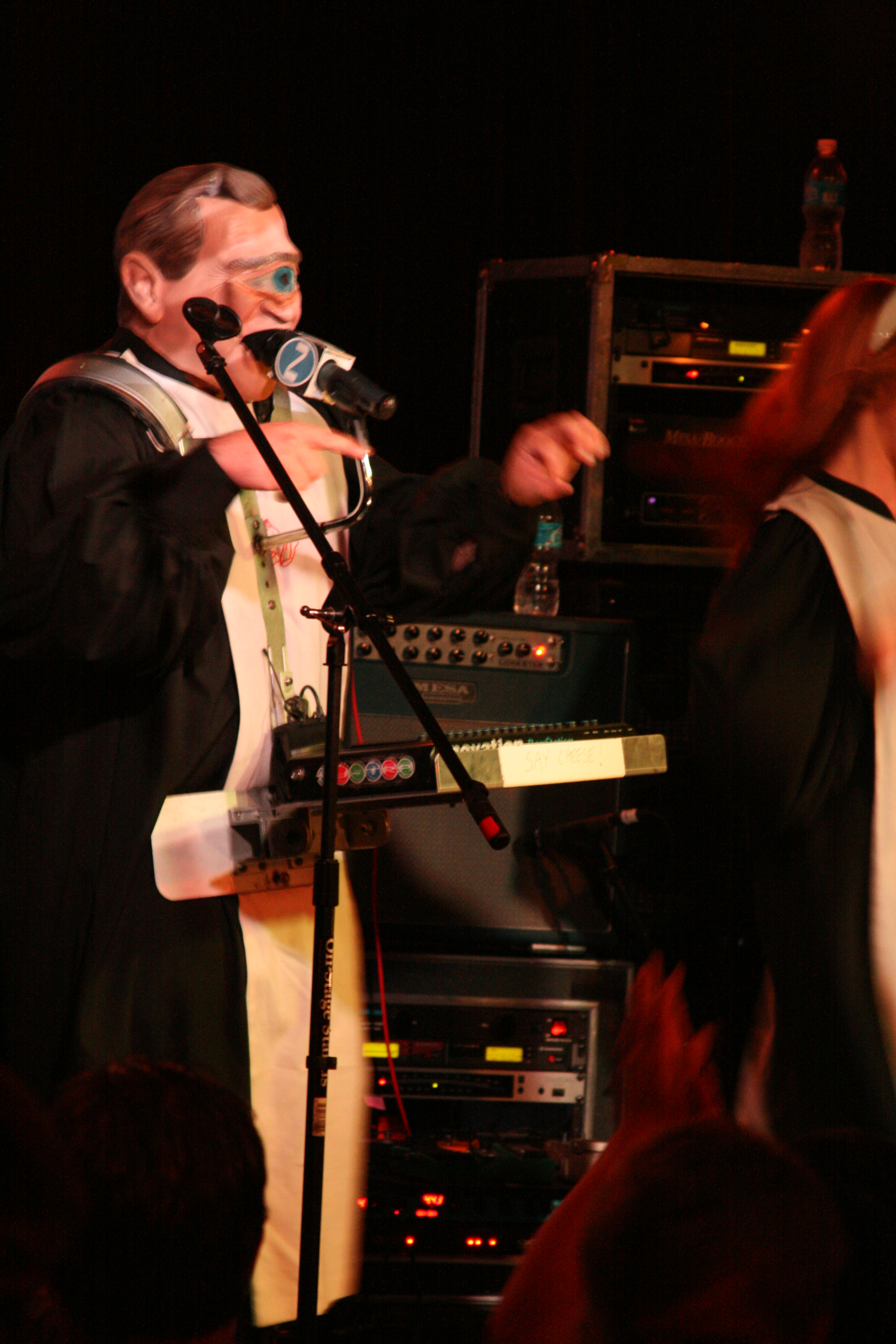#### PHOTO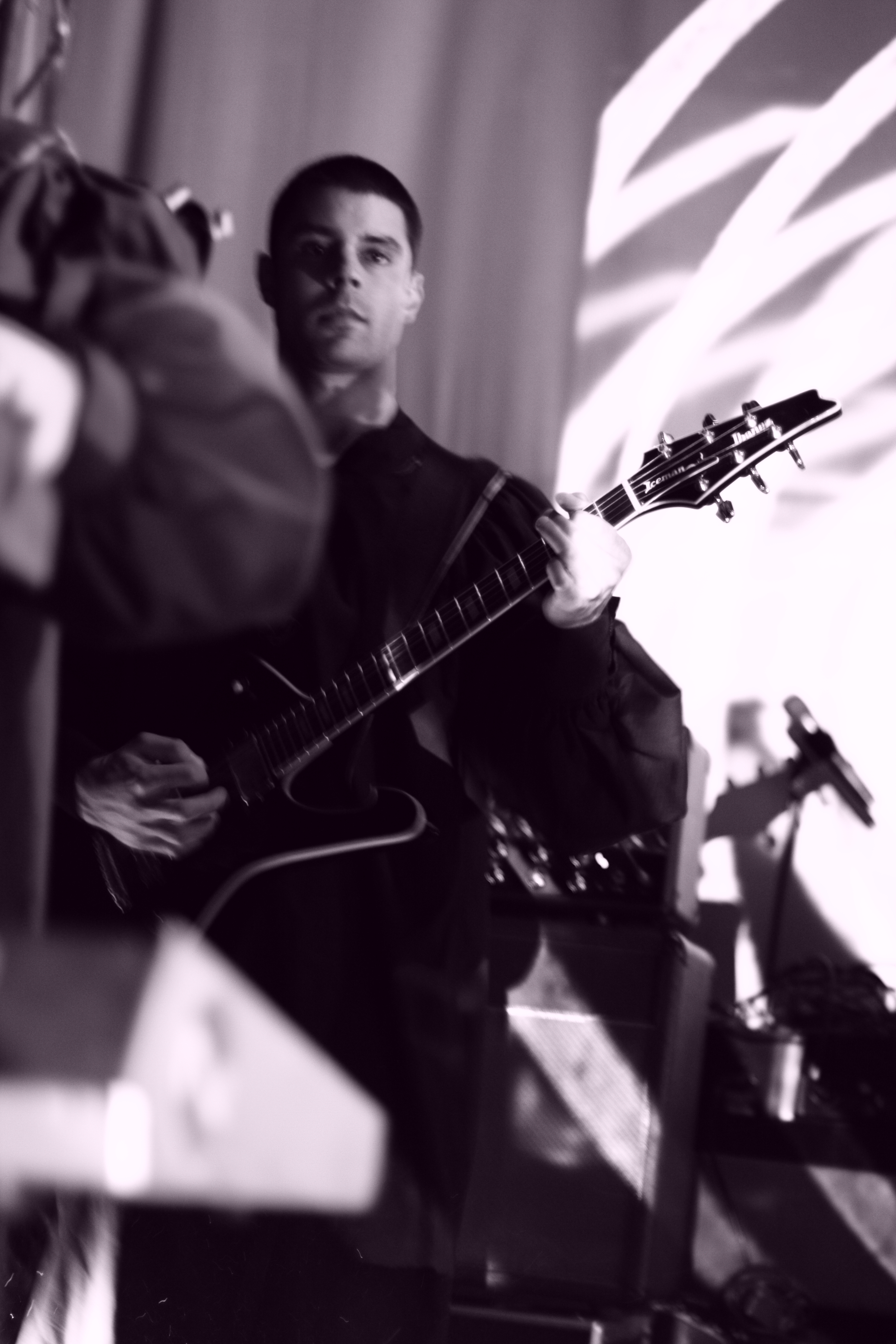### F O T O by F R A N K M O N T E S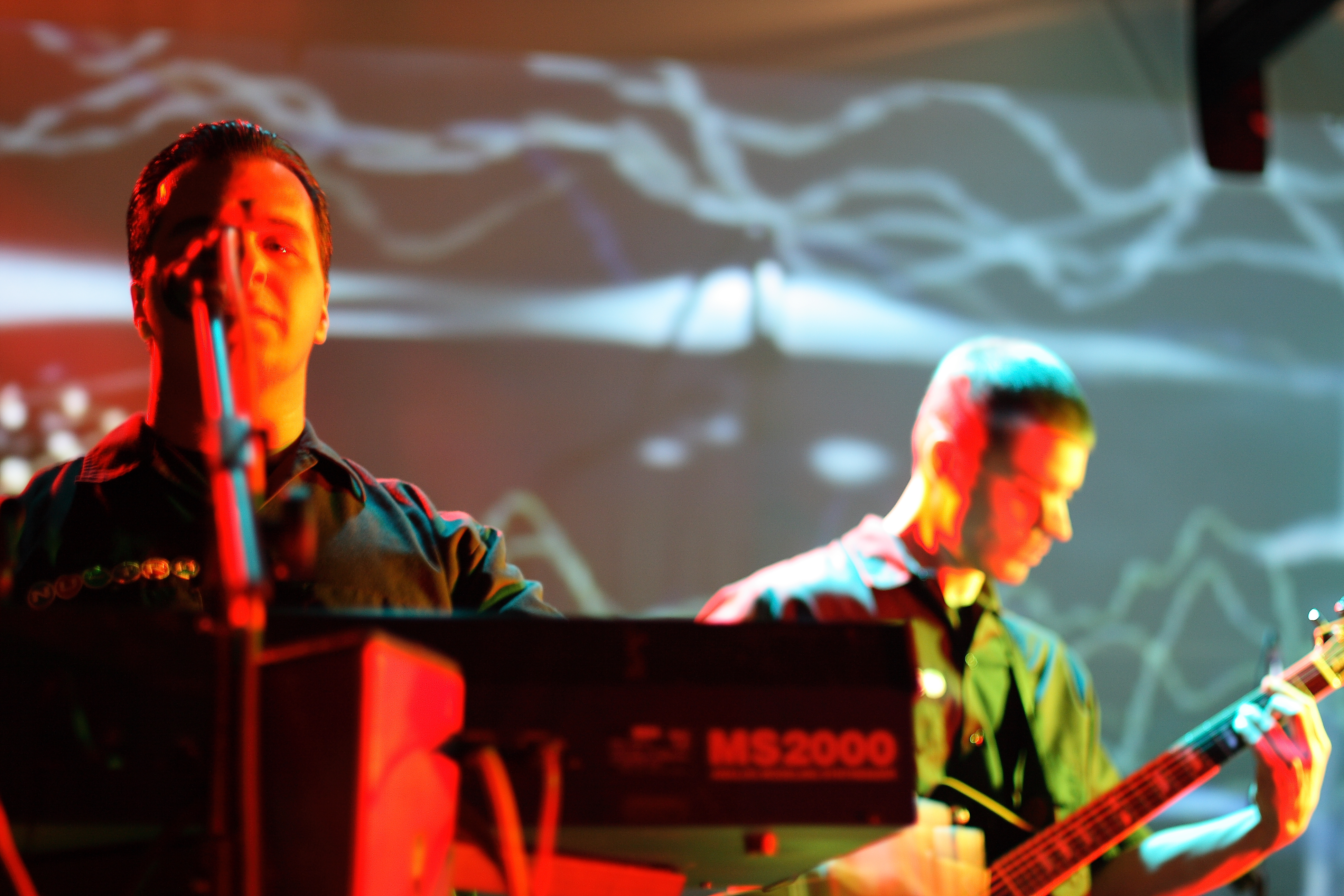### F O T O by F R A N K M O N T E S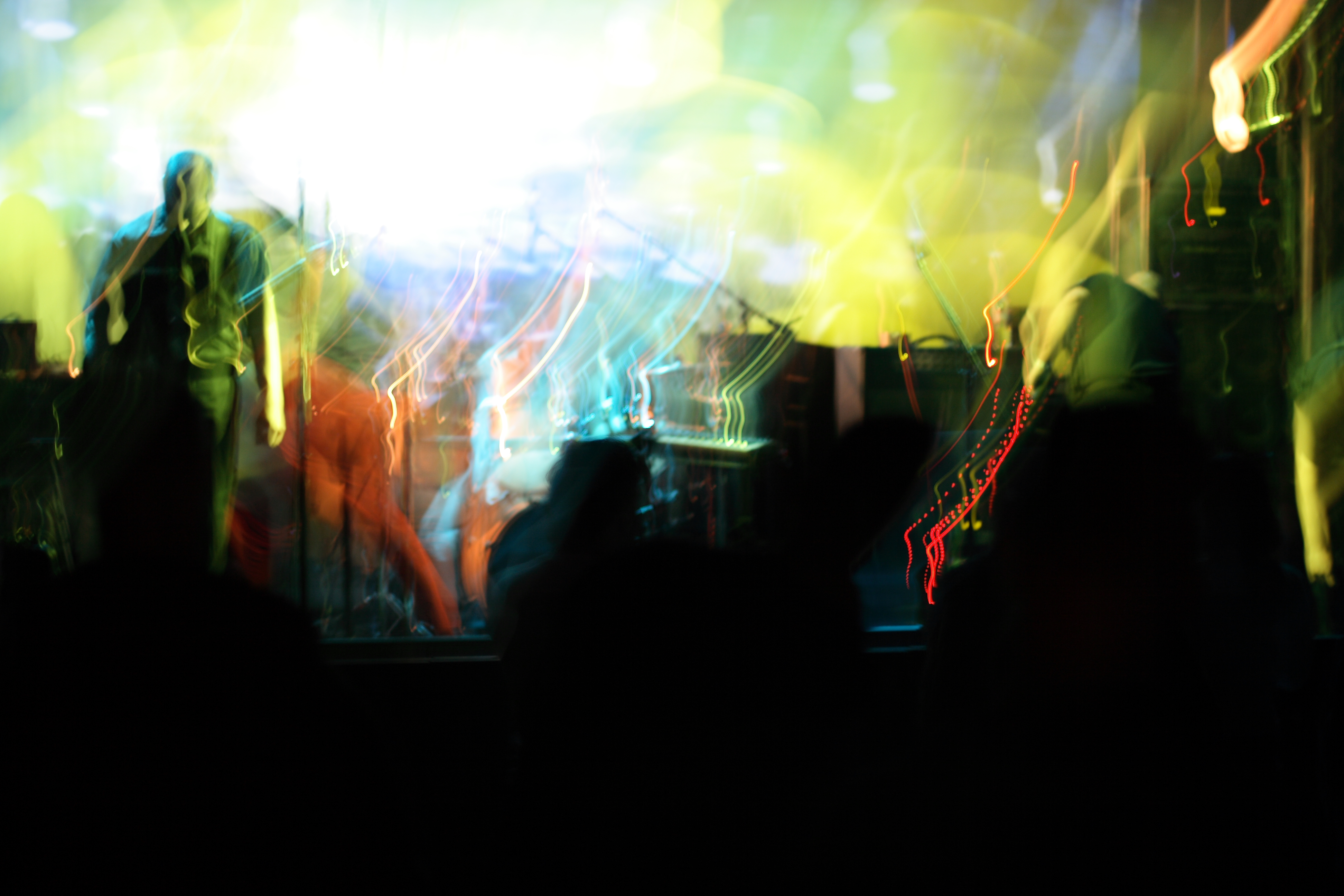### F O T O by F R A N K M O N T E S### F O T O by F R A N K M O N T E S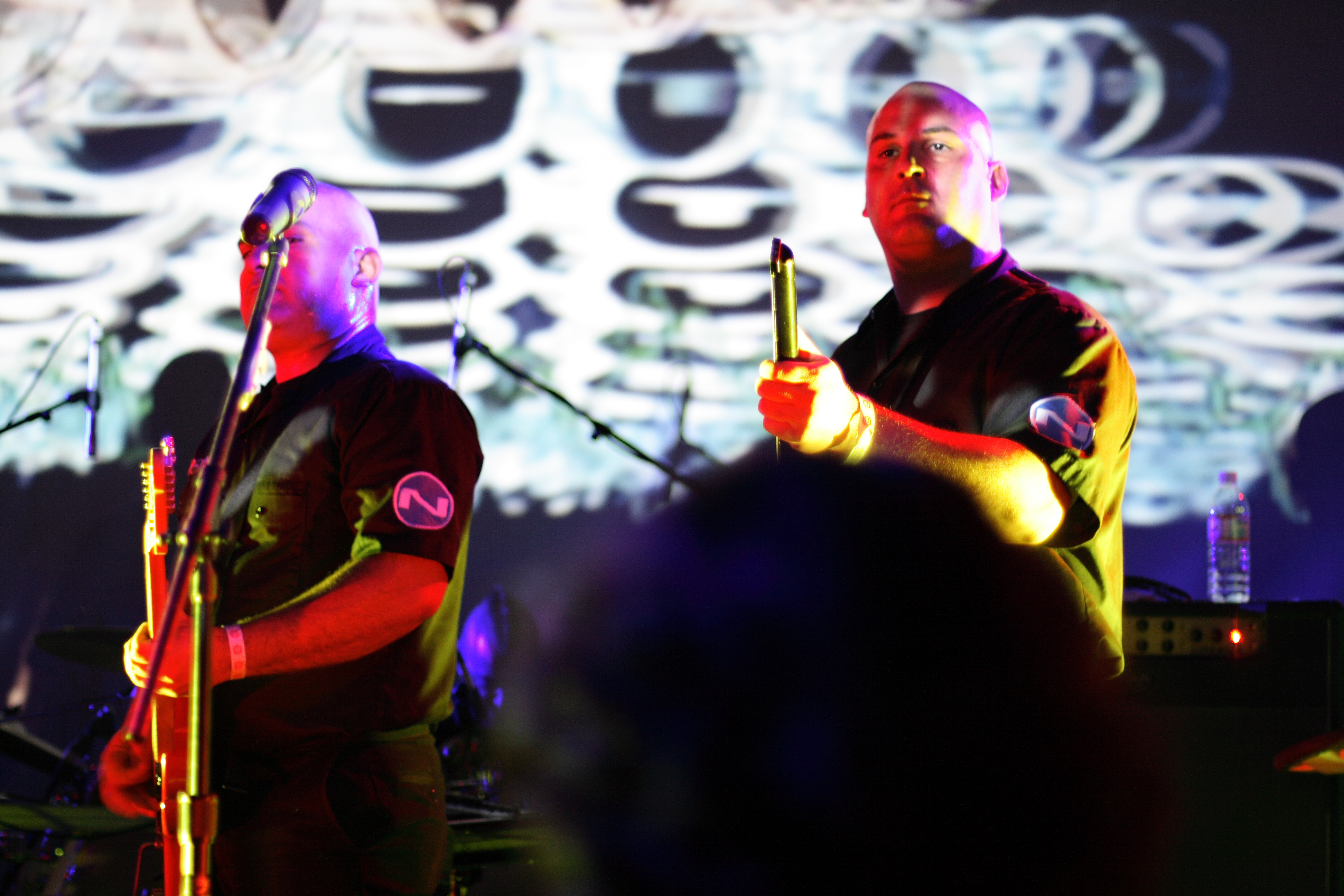### F O T O by F R A N K M O N T E S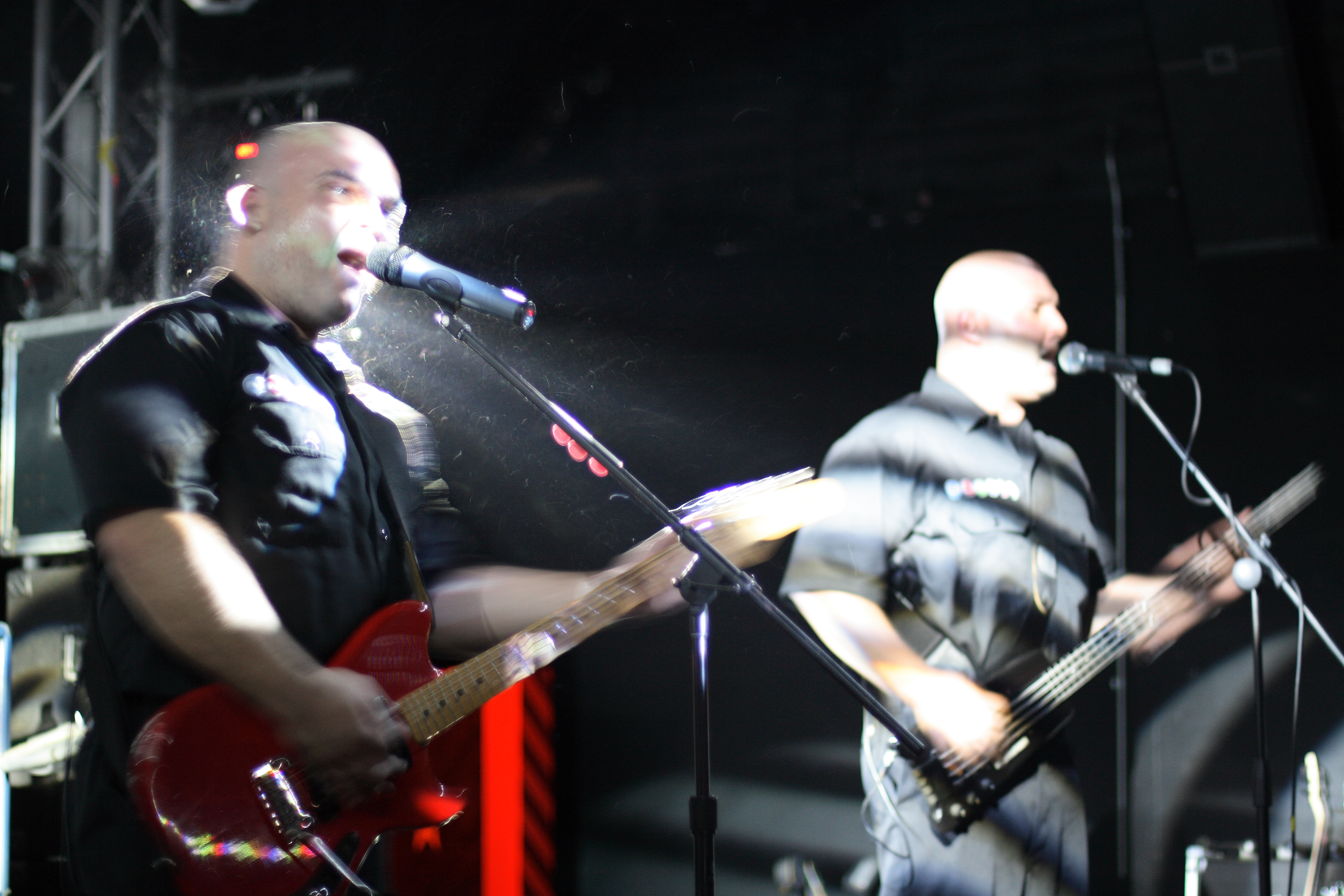### F O T O by F R A N K M O N T E S### F O T O by F R A N K M O N T E S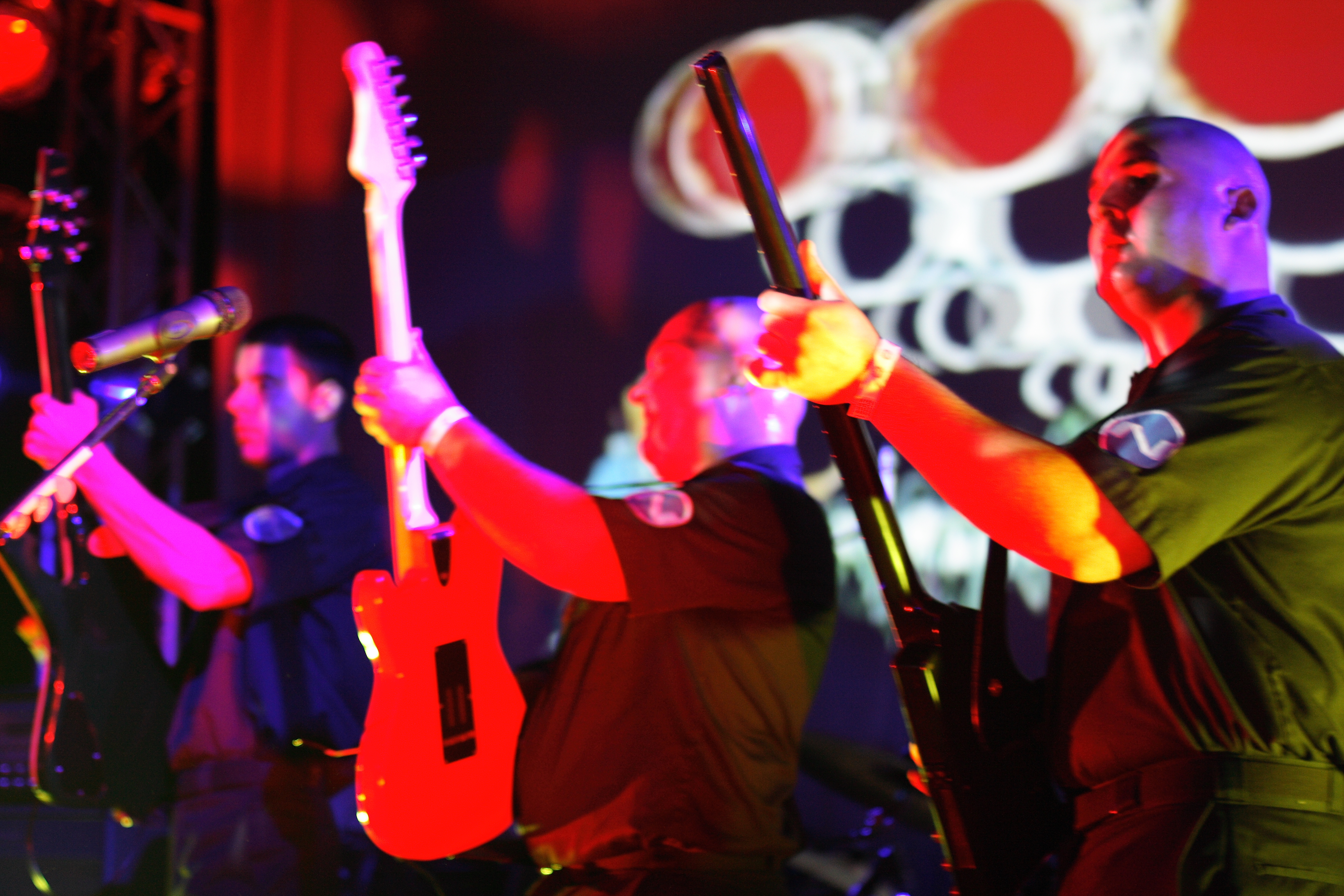### F O T O by F R A N K M O N T E S### F O T O by F R A N K M O N T E S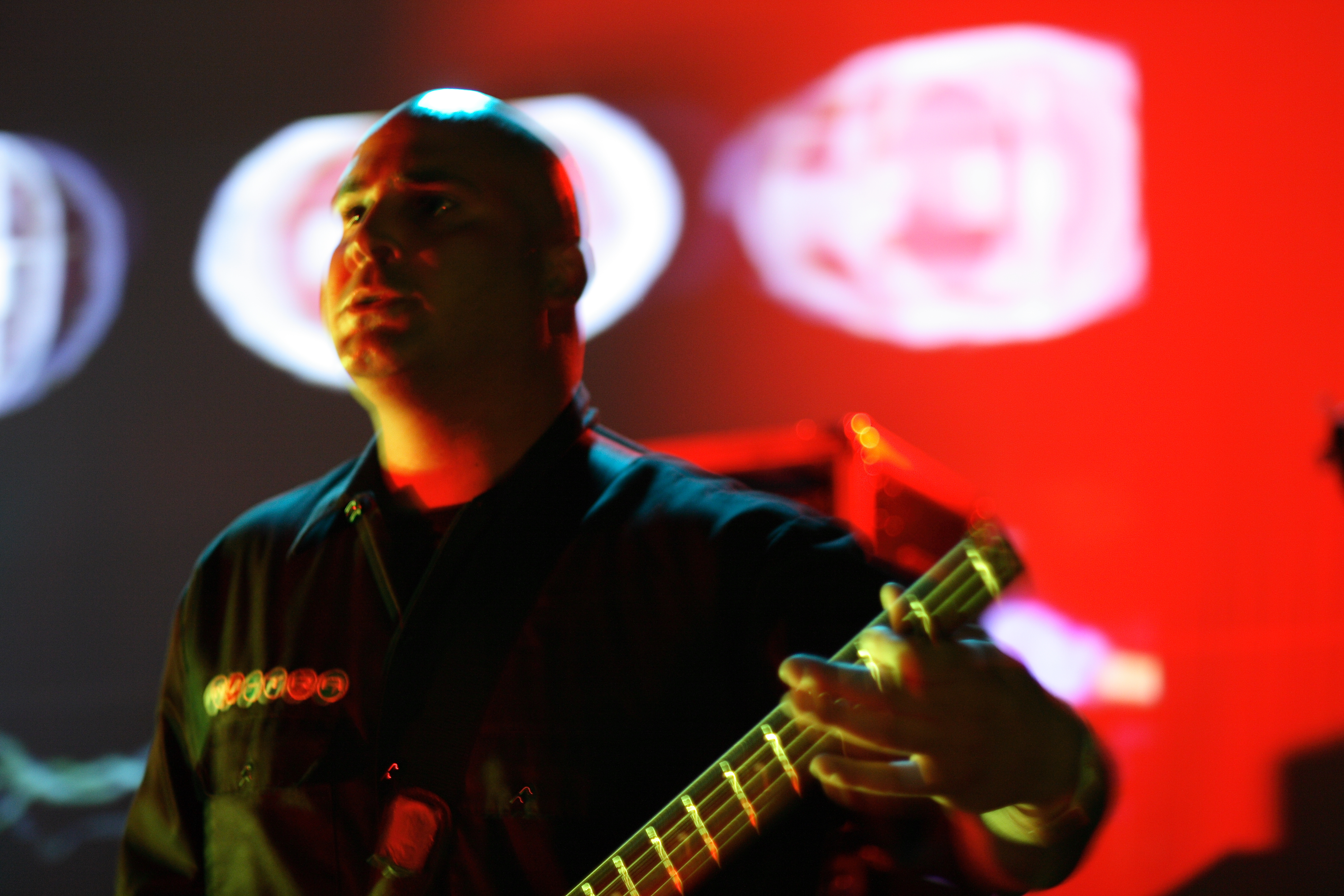### F O T O by F R A N K M O N T E S### F O T O by F R A N K M O N T E S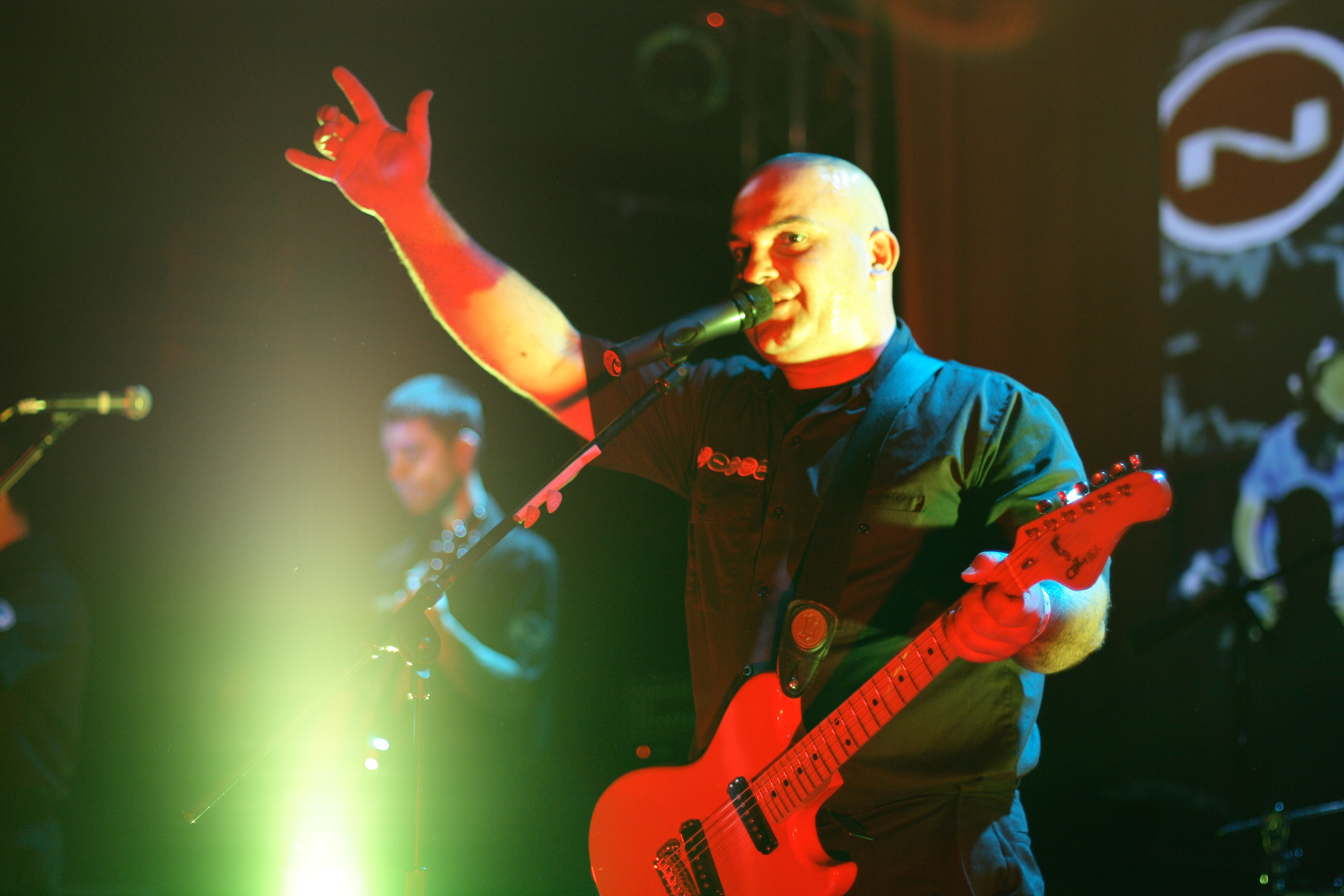### F O T O by F R A N K M O N T E S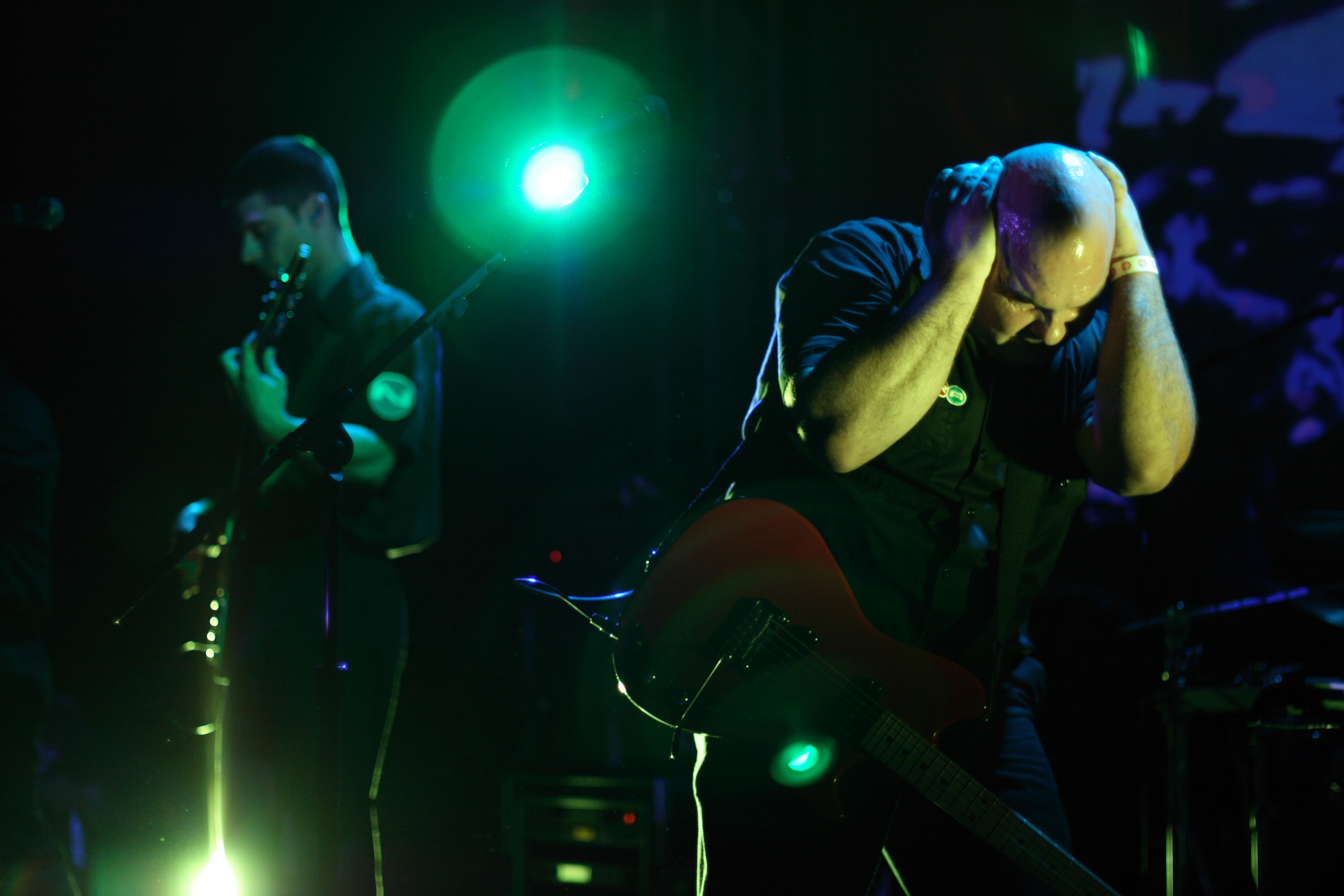### F O T O by F R A N K M O N T E S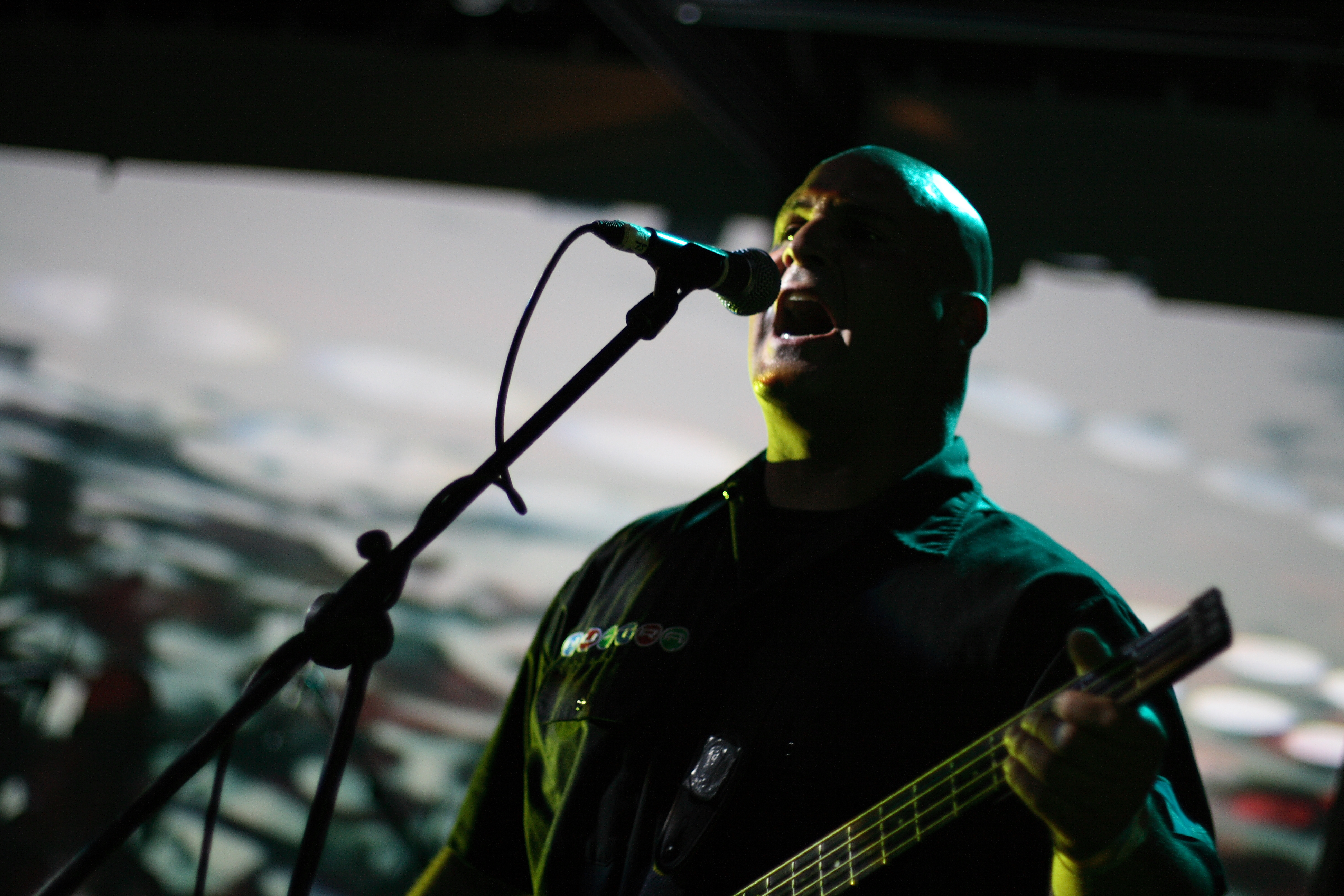### F O T O by F R A N K M O N T E S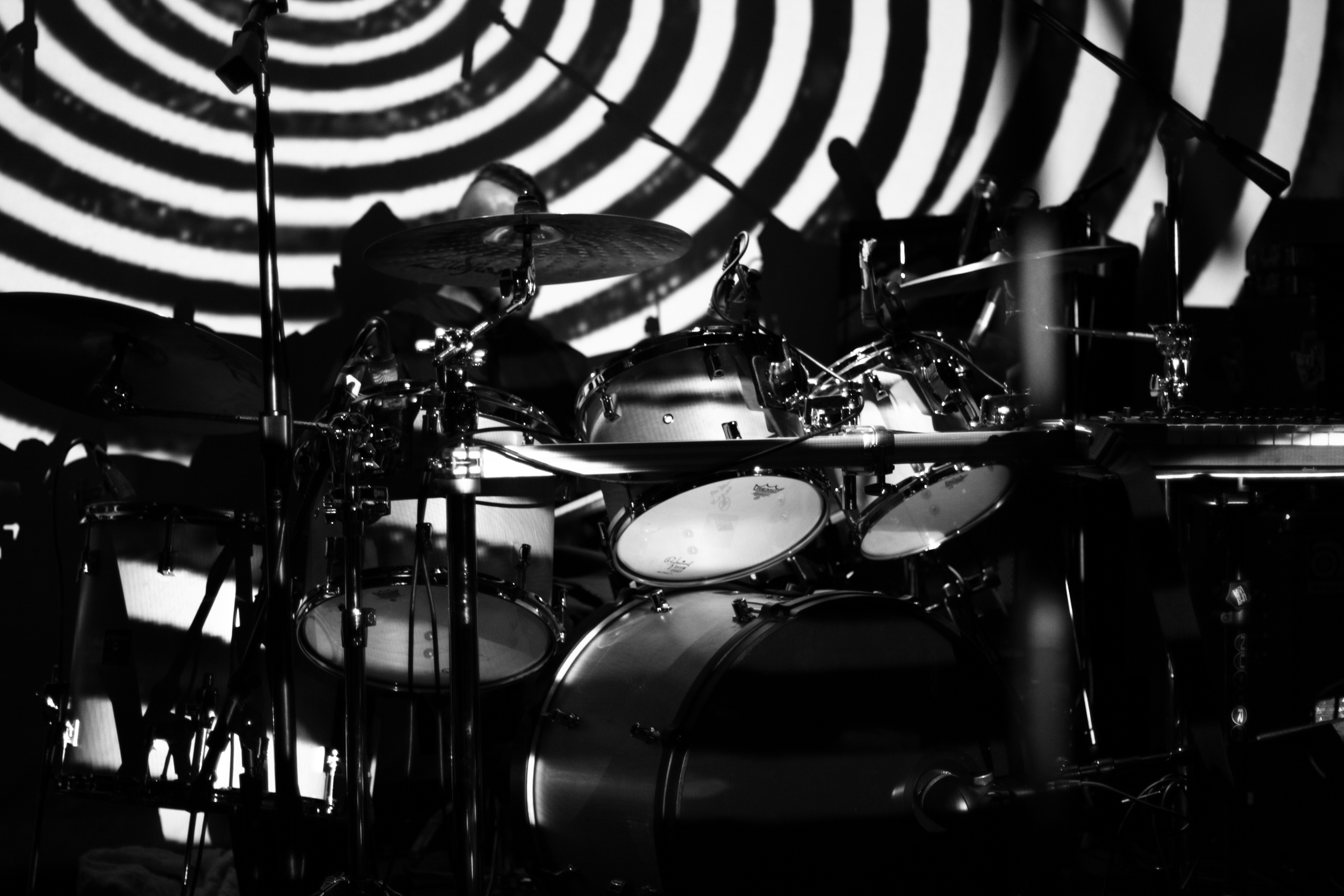### F O T O by F R A N K M O N T E S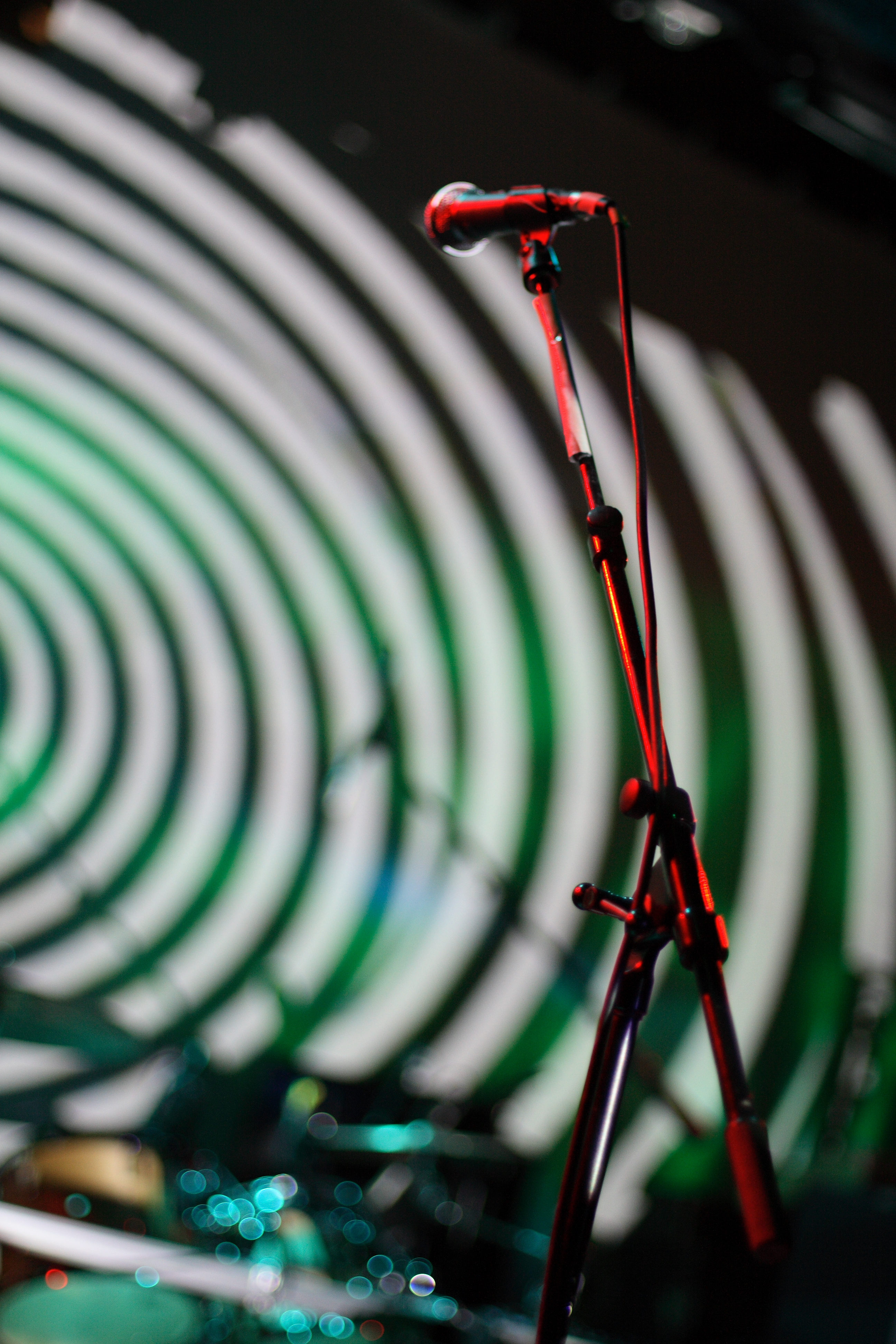### F O T O by F R A N K M O N T E S### F O T O by F R A N K M O N T E S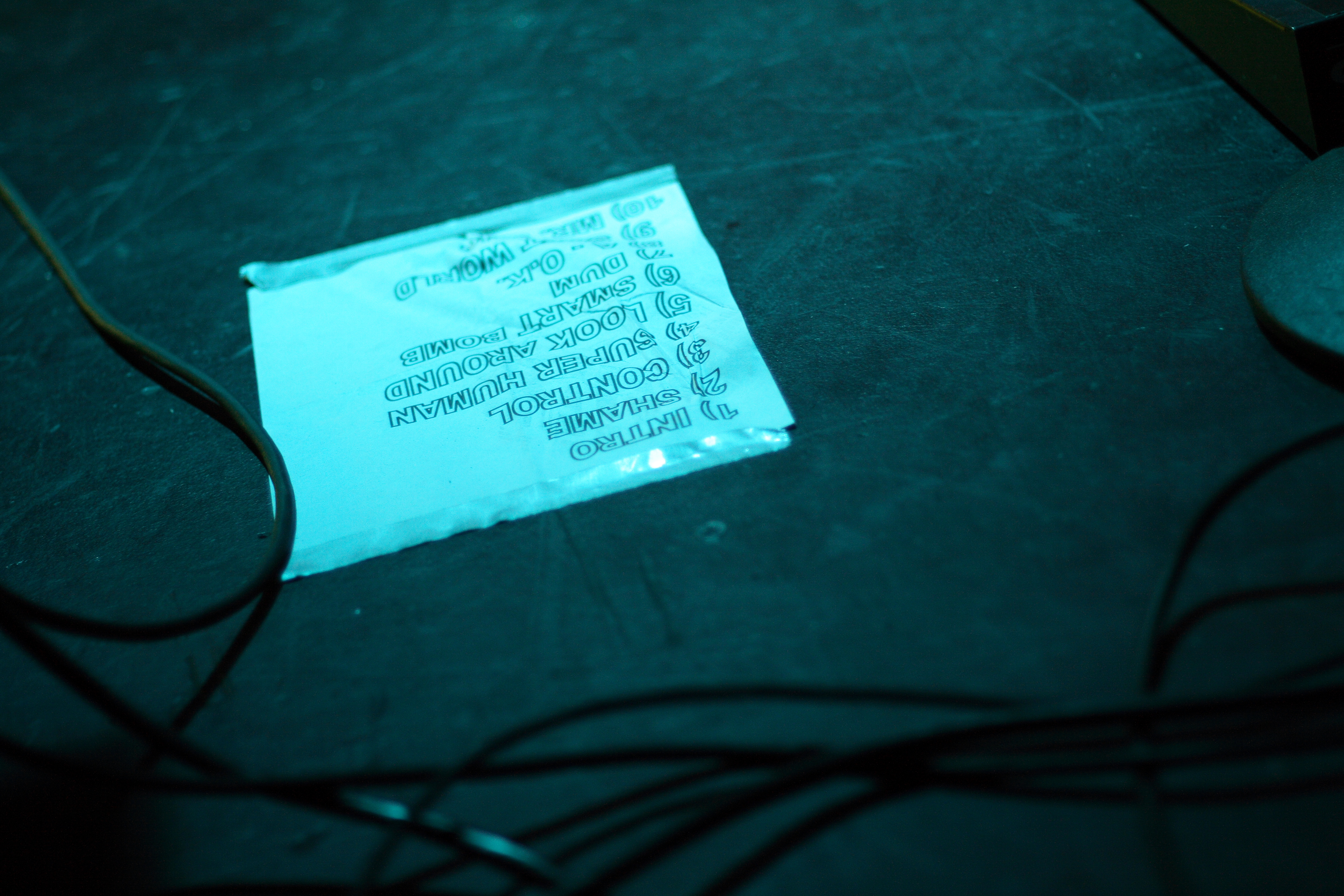### F O T O by F R A N K M O N T E S### F O T O by F R A N K M O N T E S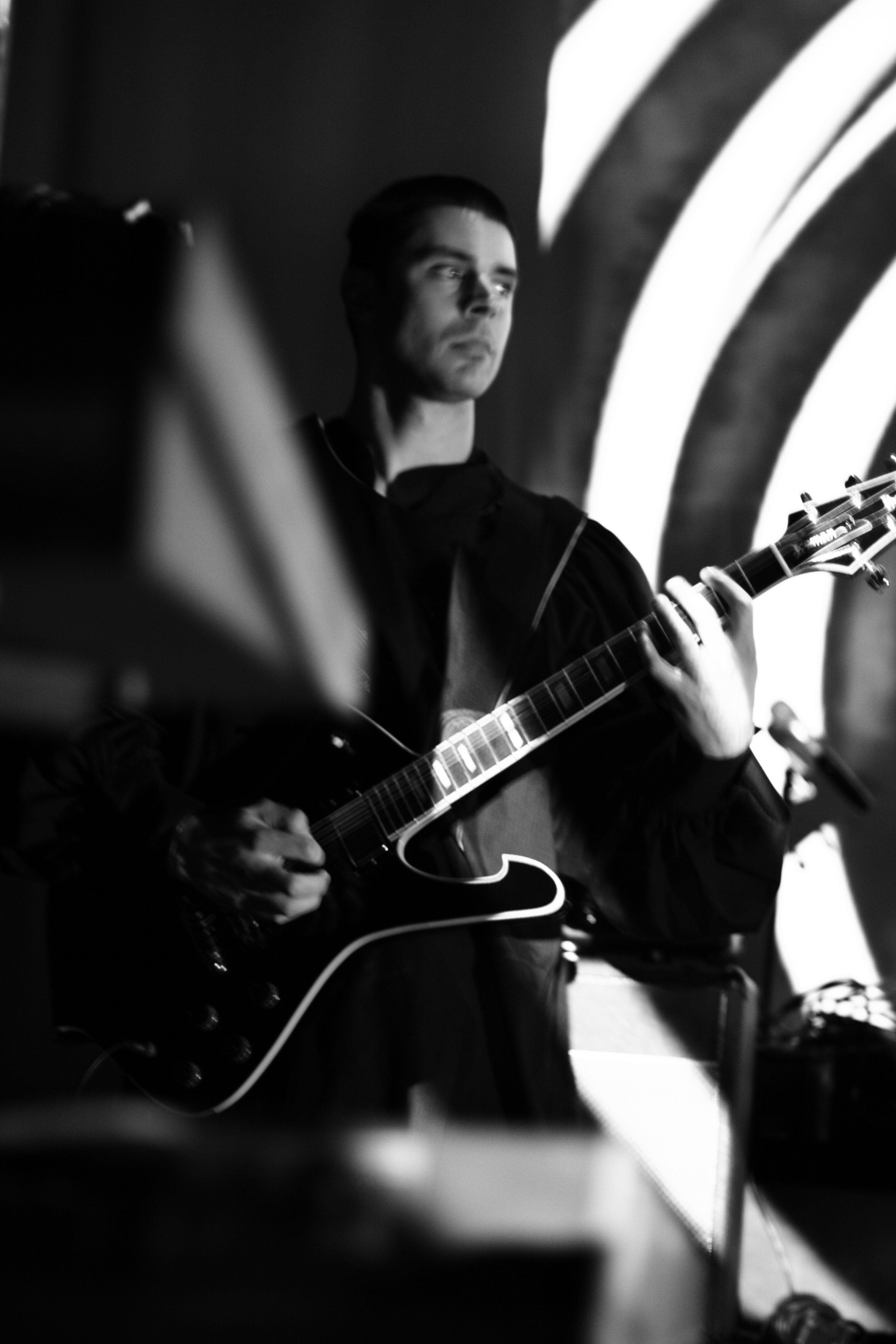### F O T O by F R A N K M O N T E S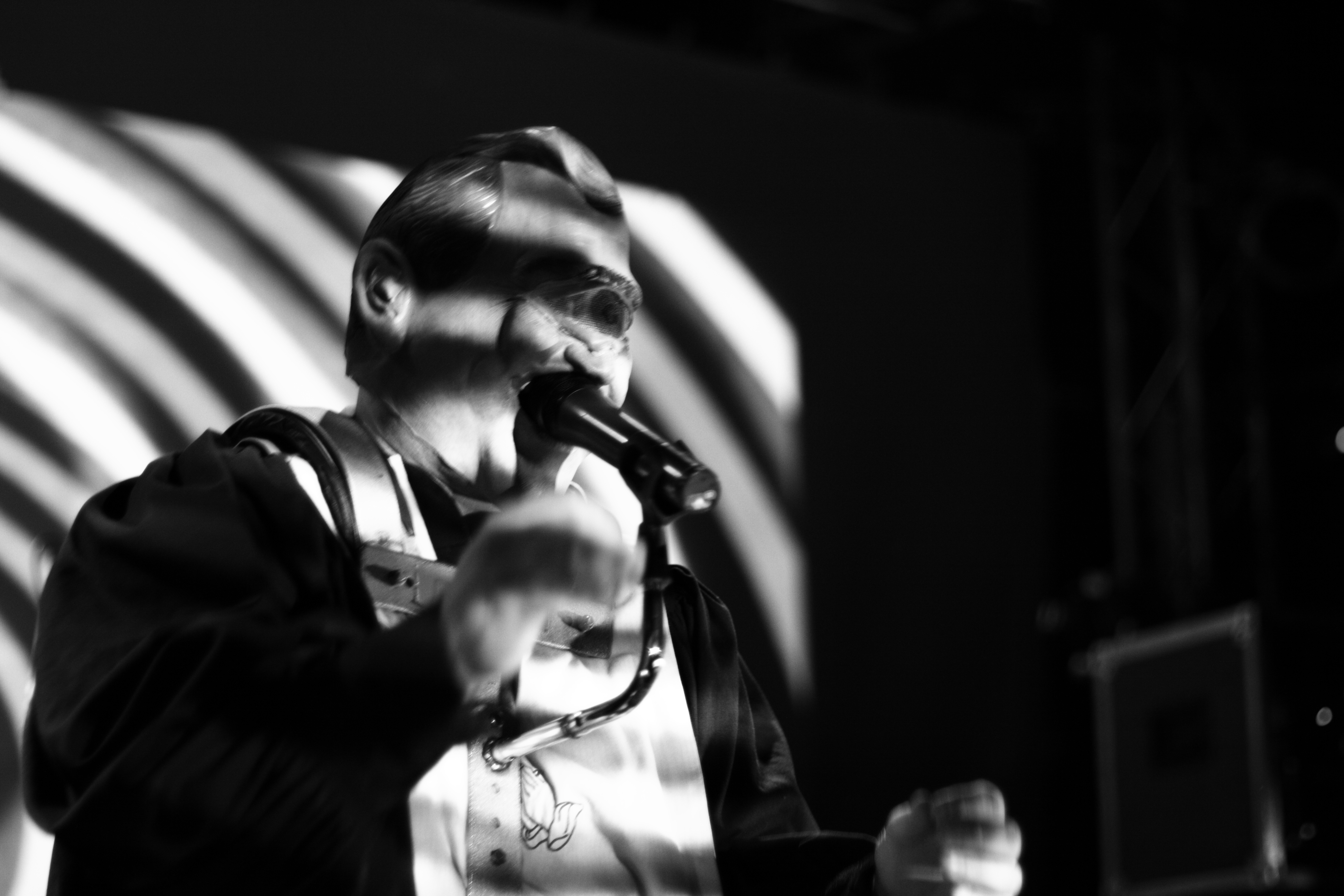### F O T O by F R A N K M O N T E S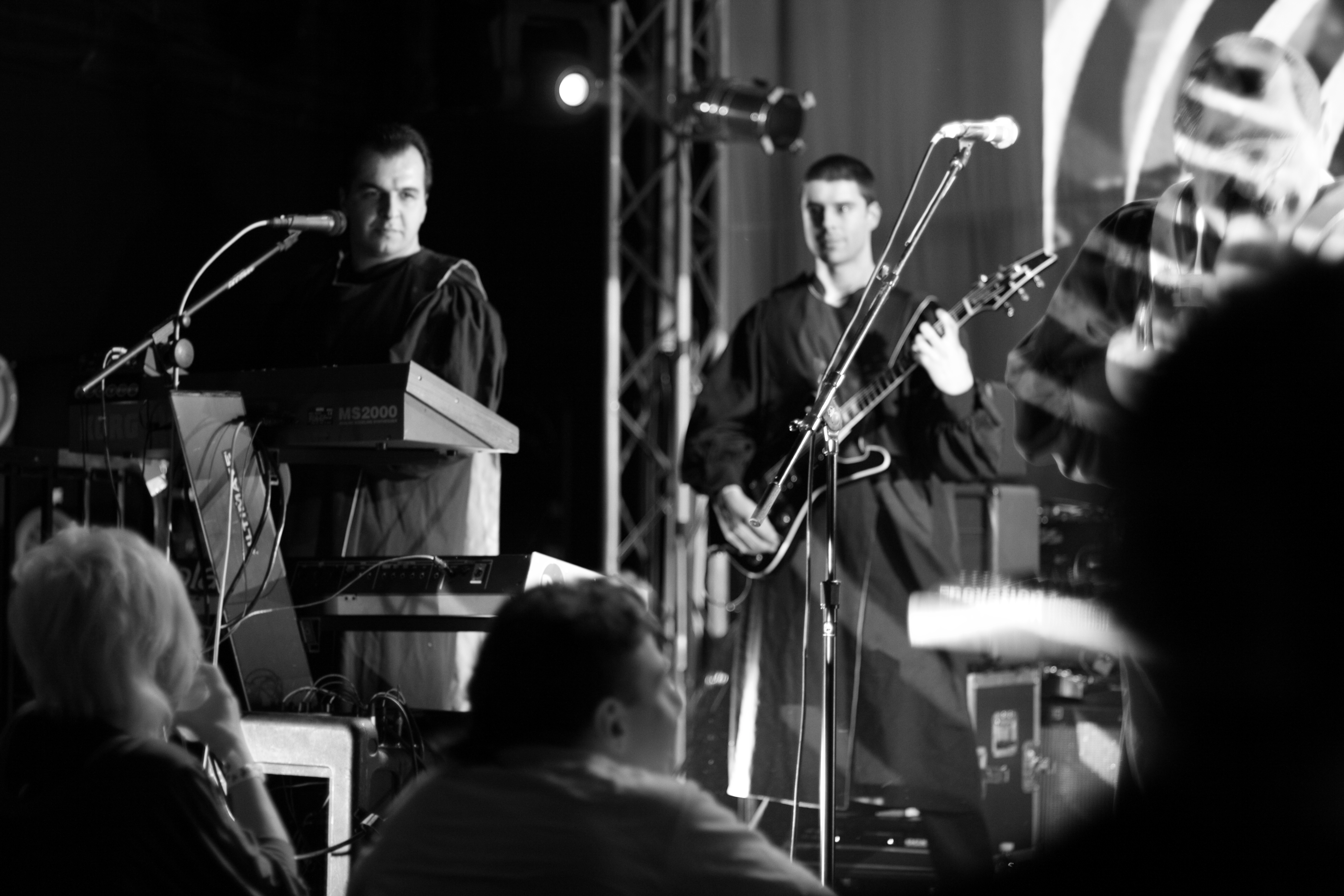### F O T O by F R A N K M O N T E S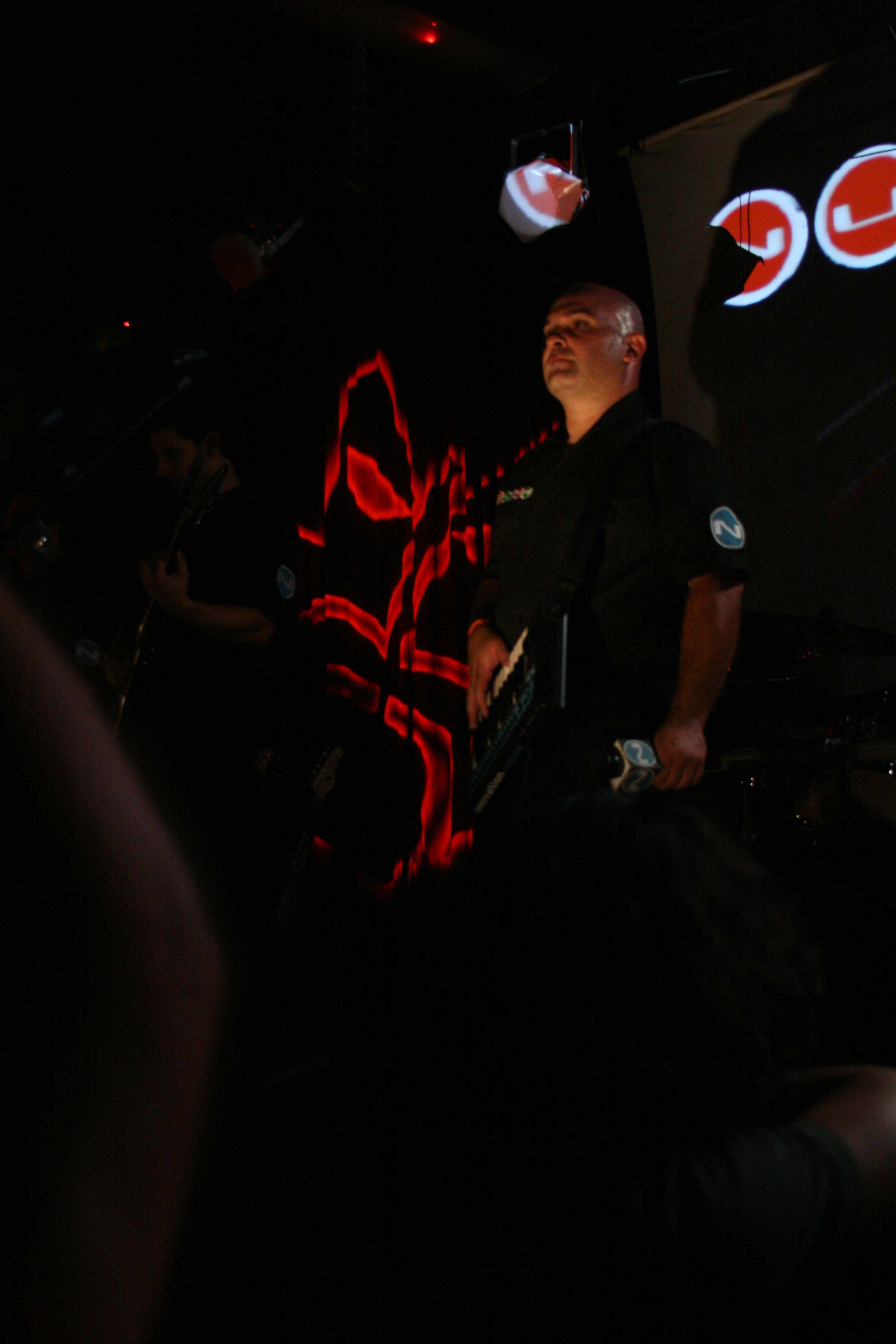### F O T O by F R A N K M O N T E S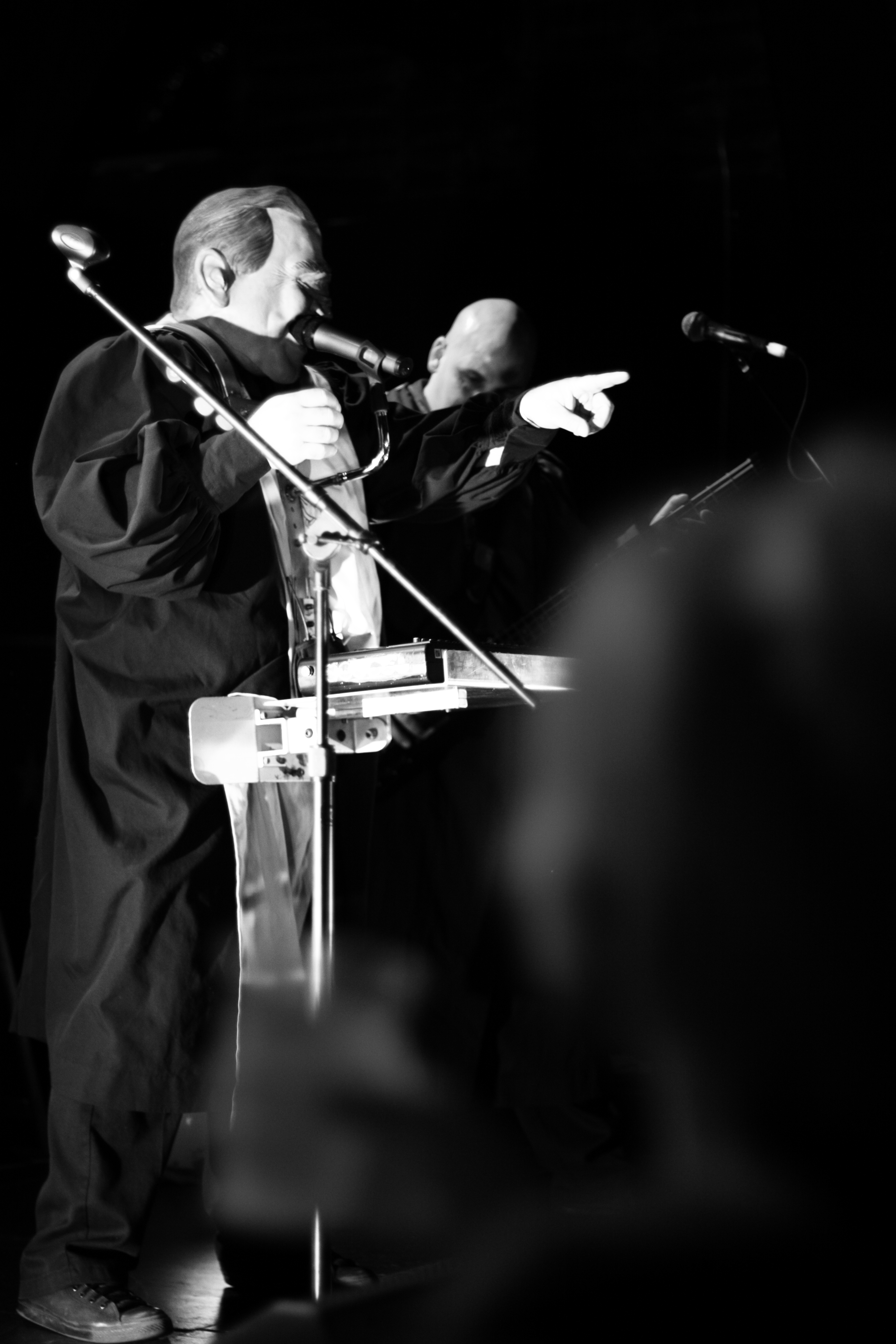### F O T O by F R A N K M O N T E S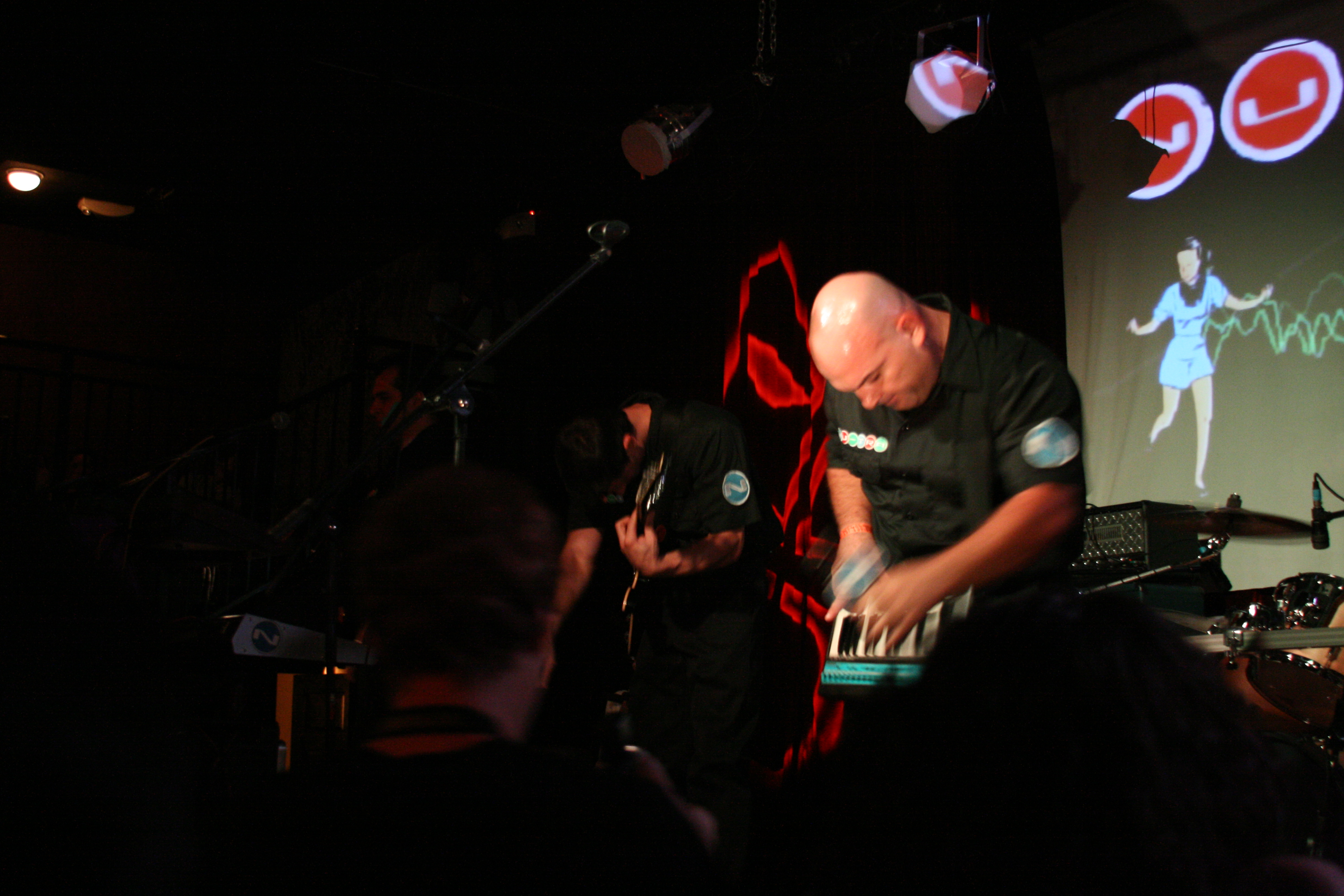### F O T O by F R A N K M O N T E S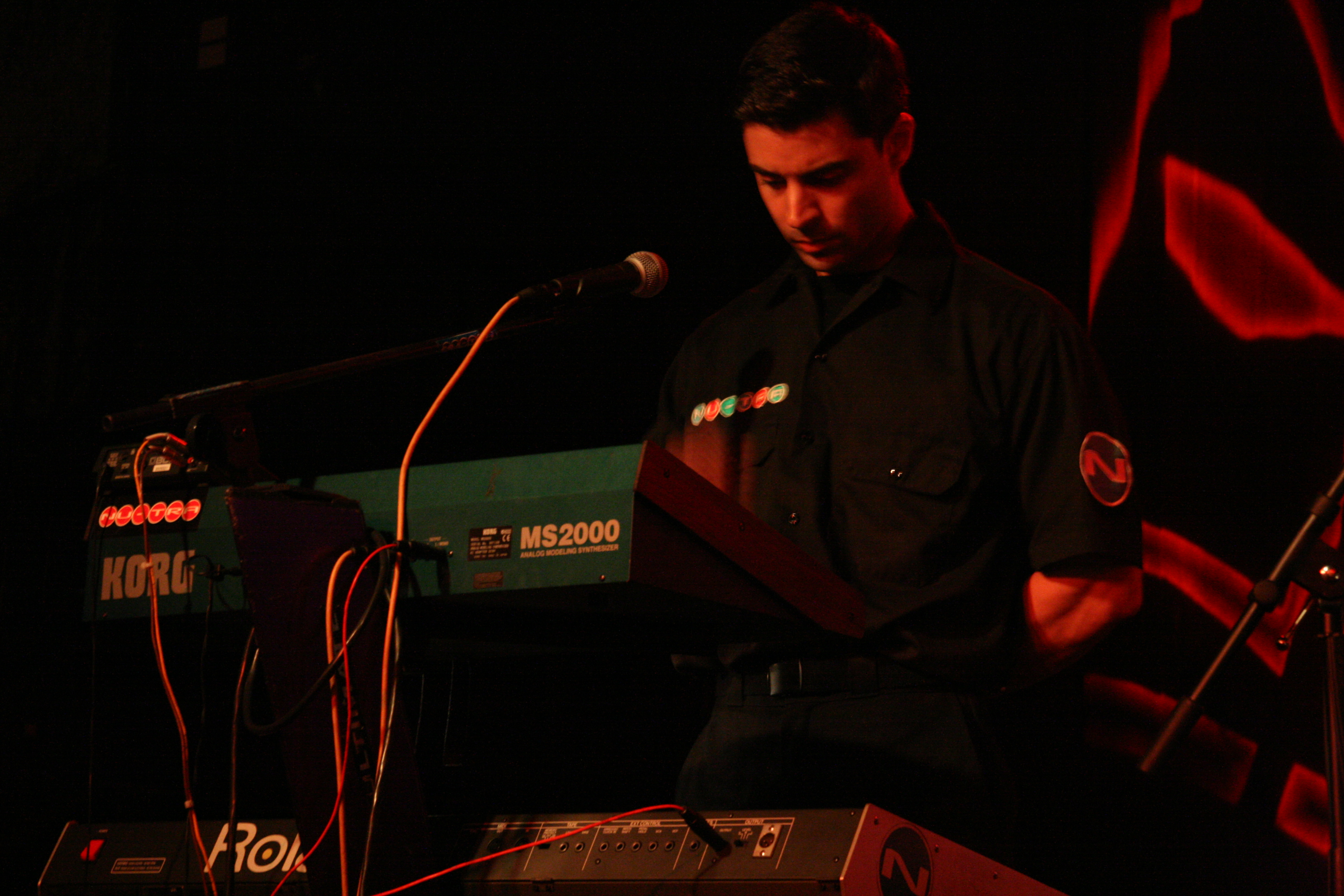### F O T O by F R A N K M O N T E S### F O T O by F R A N K M O N T E S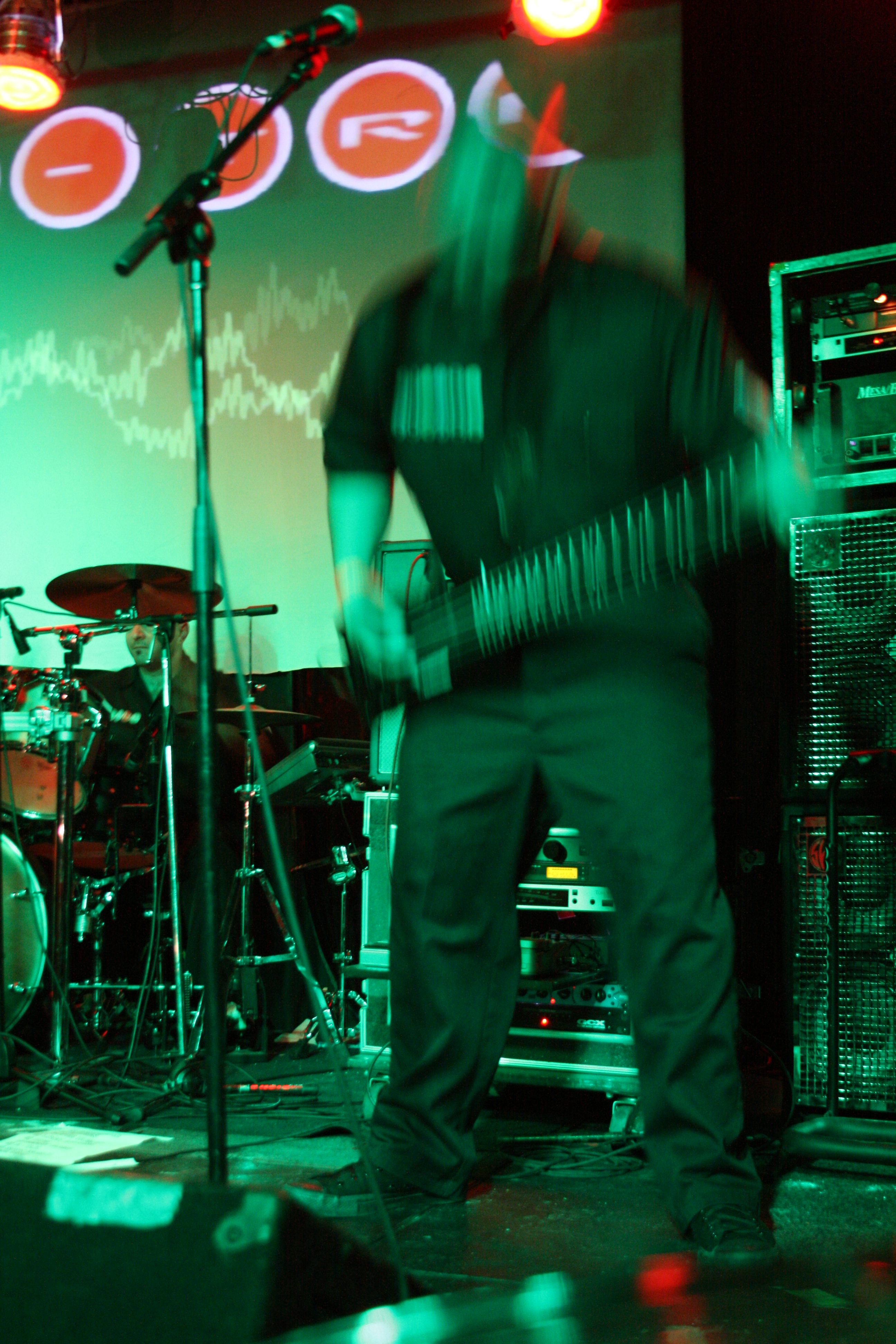### F O T O by F R A N K M O N T E S### F O T O by F R A N K M O N T E S### F O T O by F R A N K M O N T E S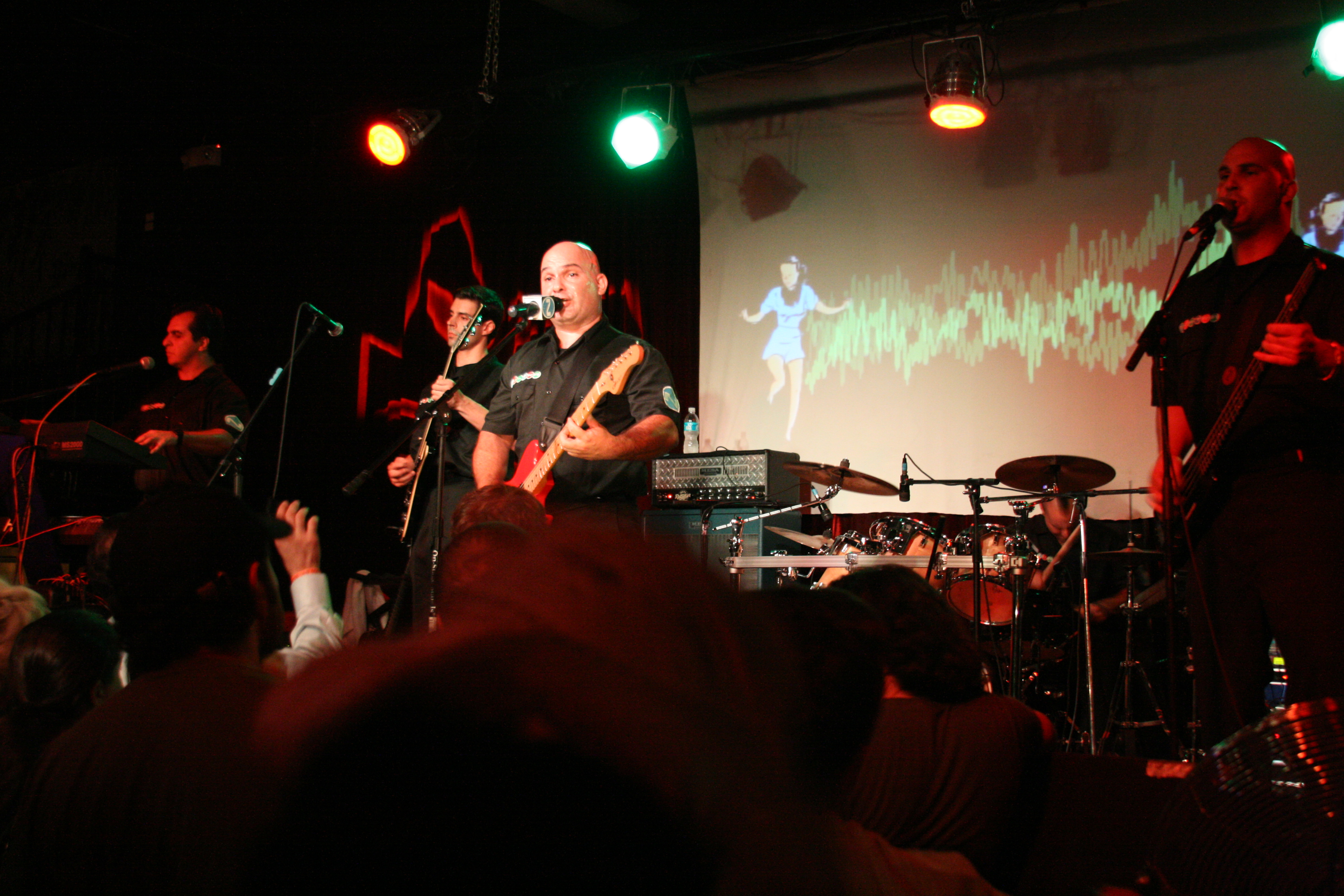### F O T O by F R A N K M O N T E S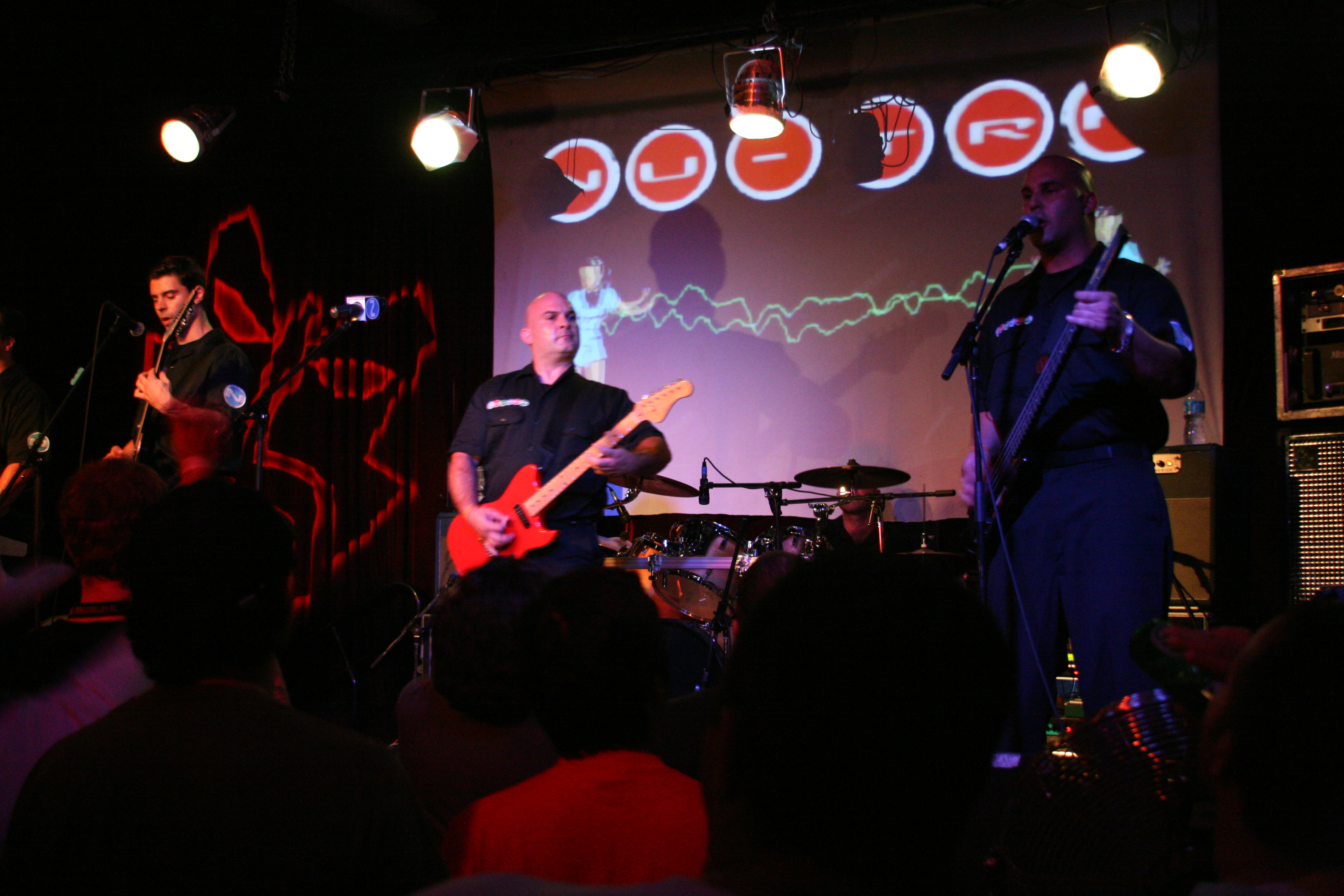### F O T O by F R A N K M O N T E S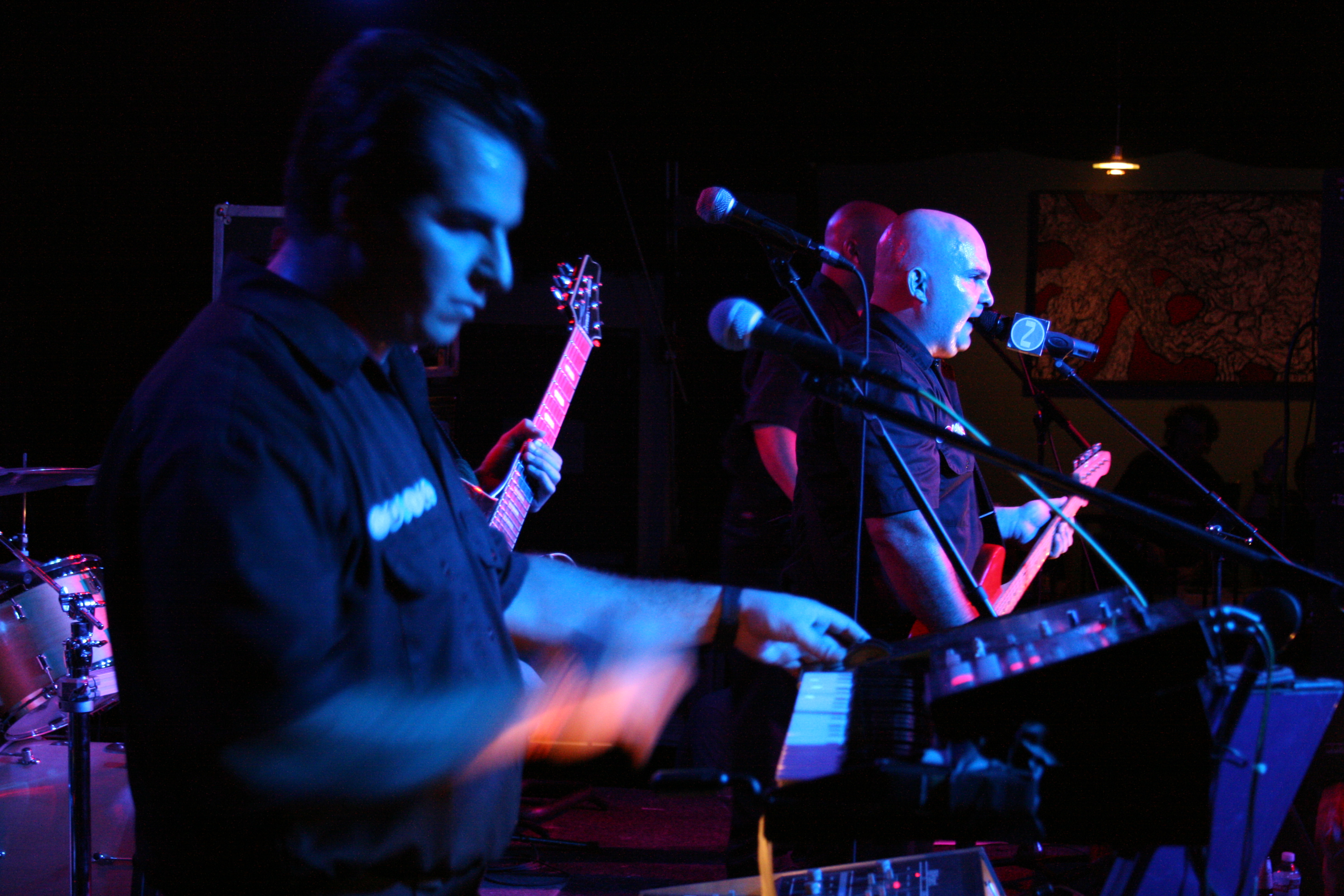### F O T O by F R A N K M O N T E S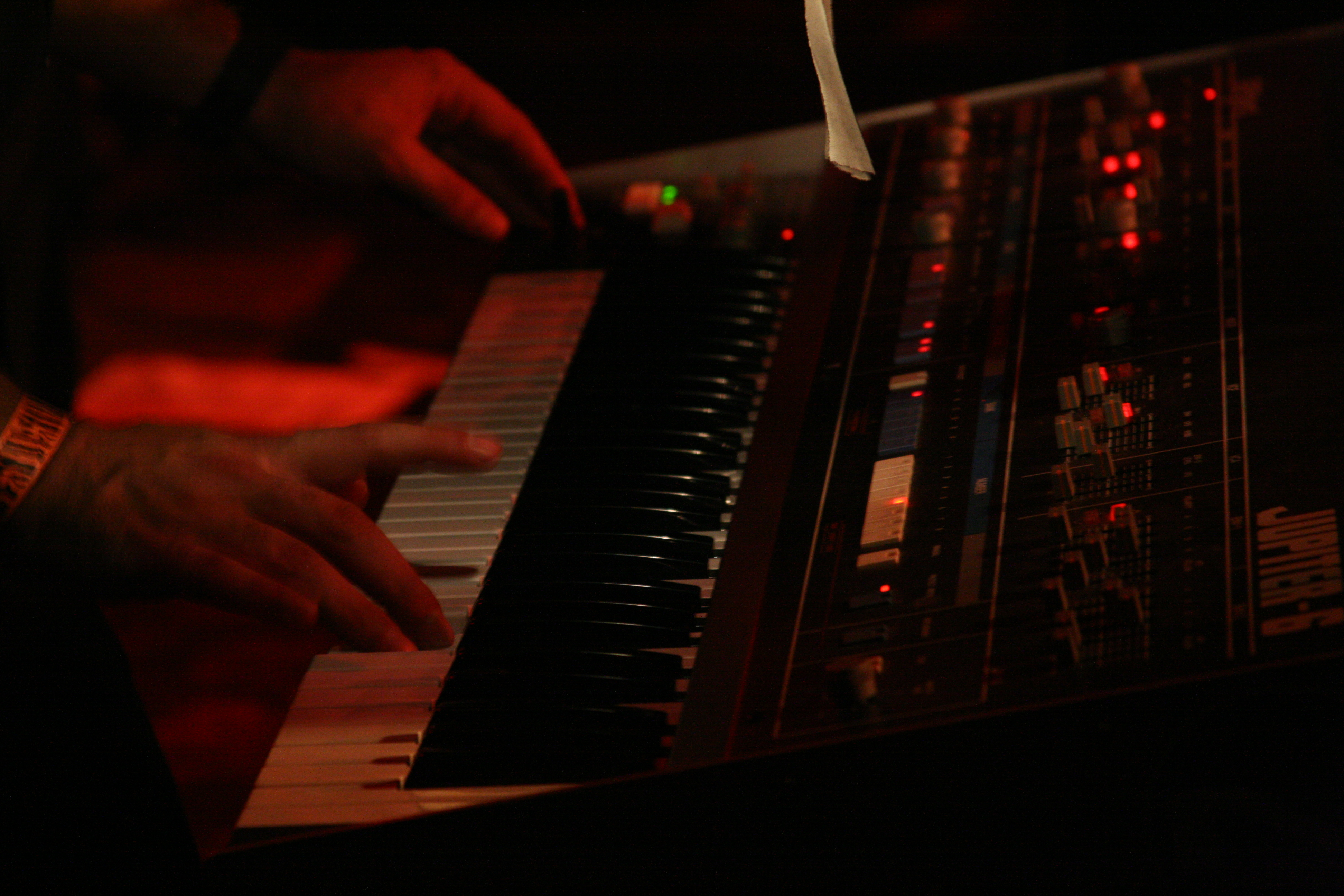### F O T O by F R A N K M O N T E S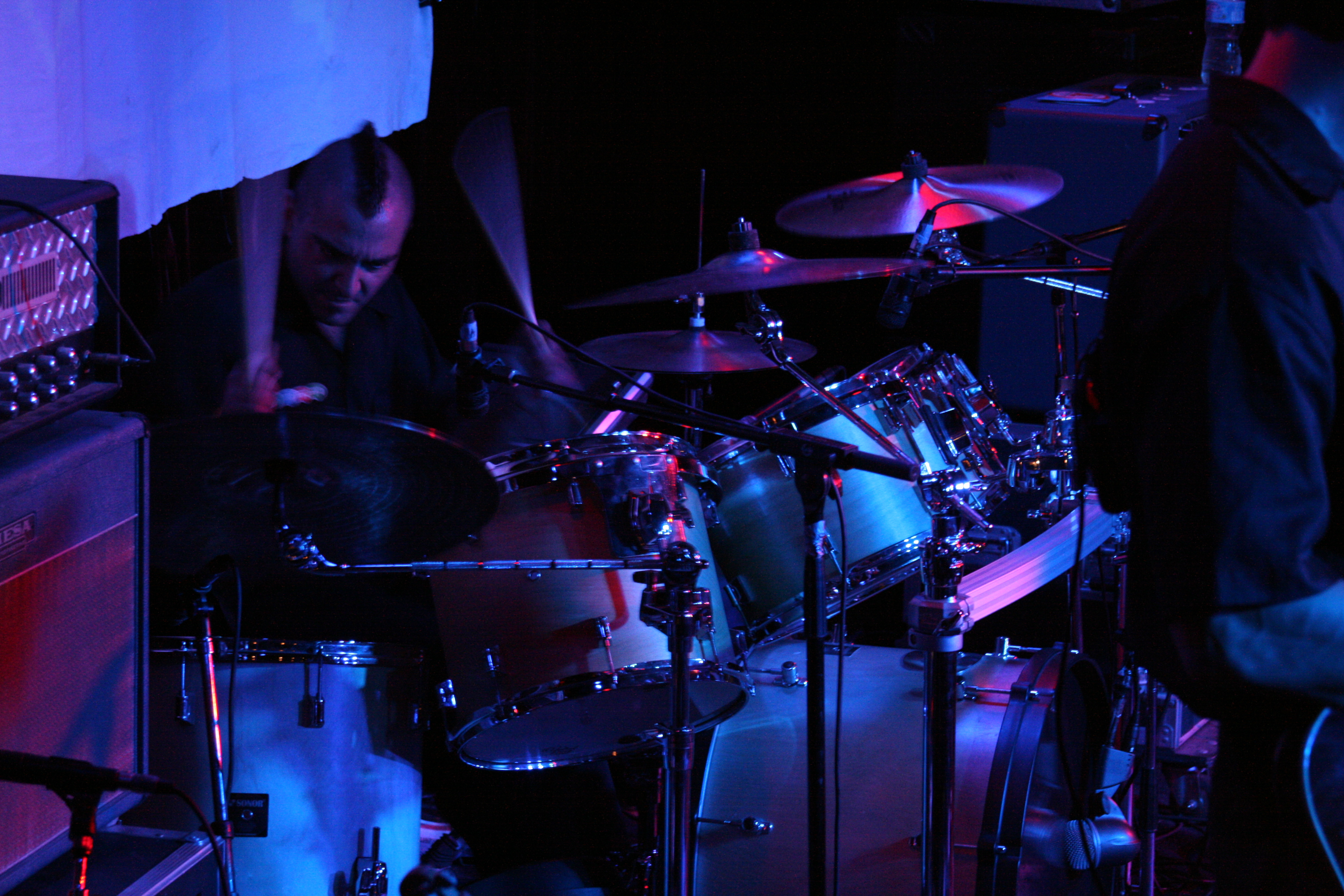### F O T O by F R A N K M O N T E S### F O T O by F R A N K M O N T E S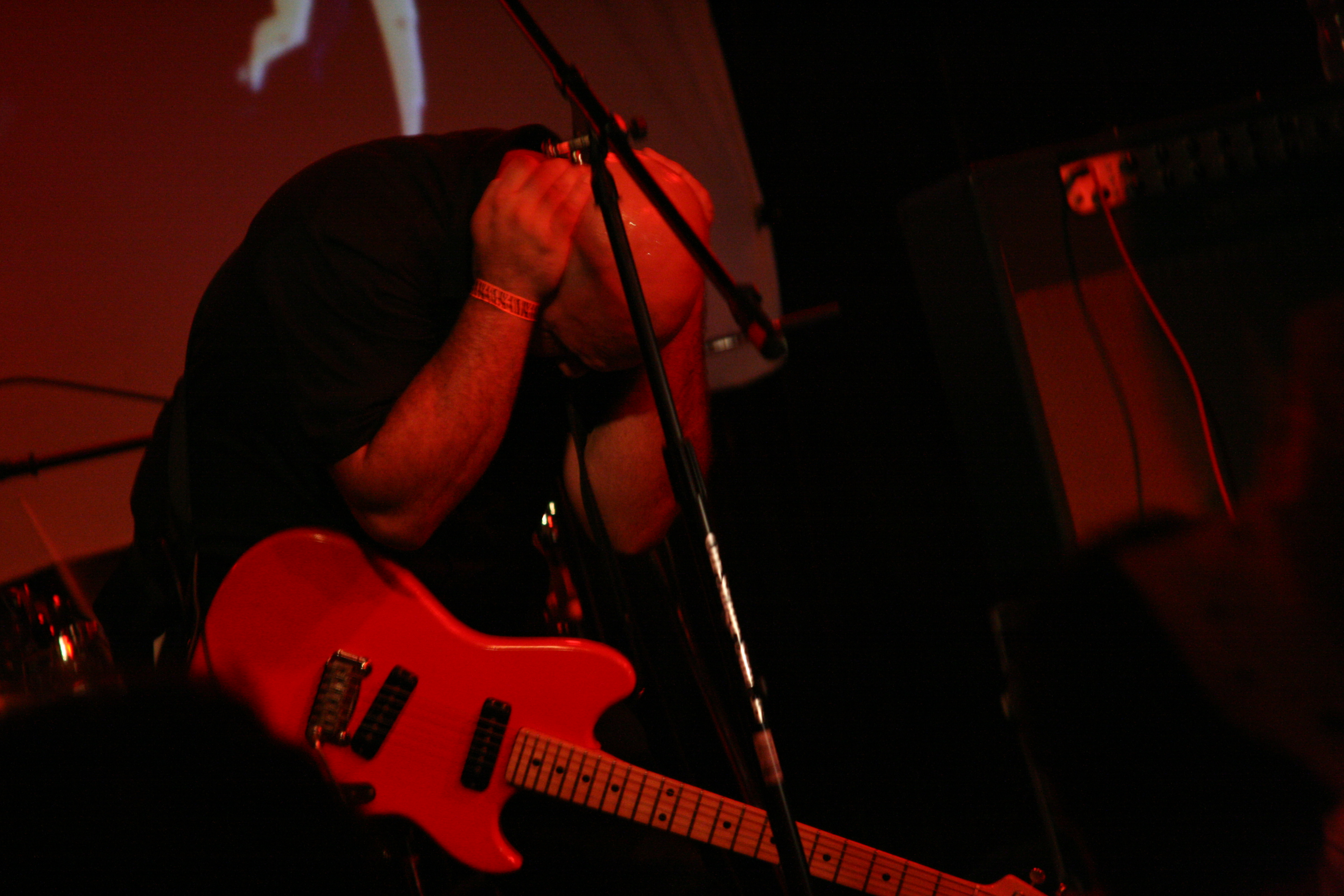### F O T O by F R A N K M O N T E S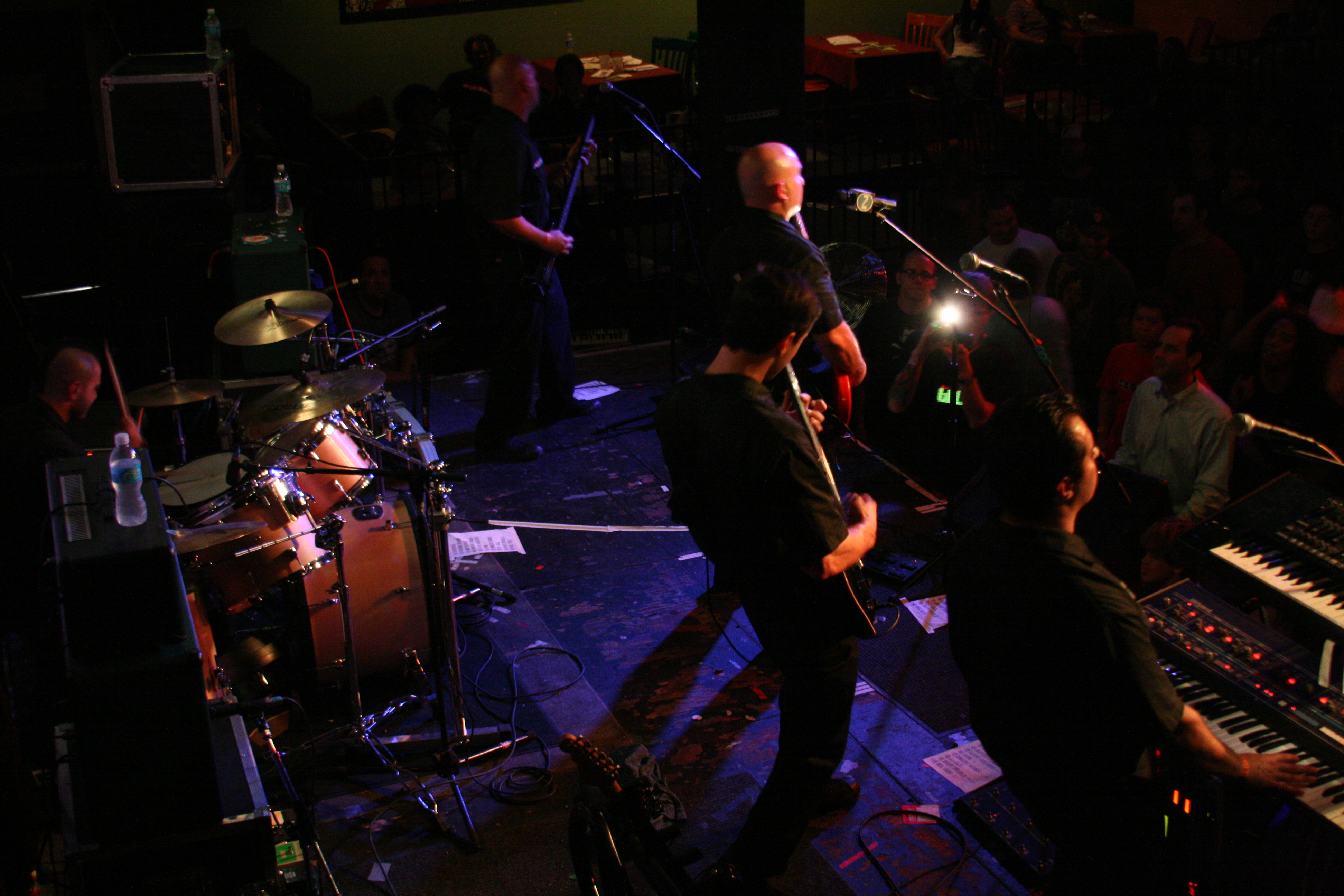### F O T O by F R A N K M O N T E S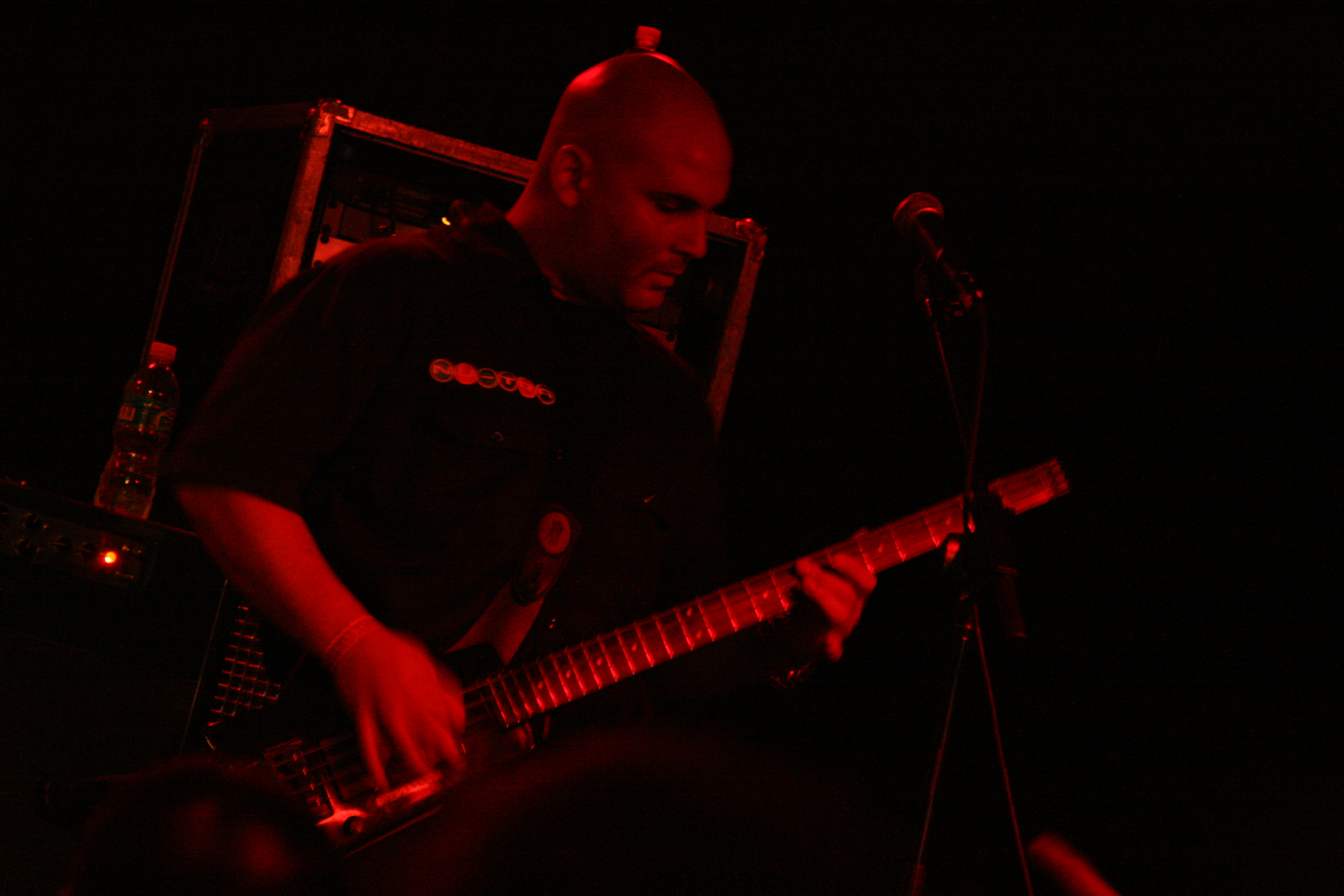### F O T O by F R A N K M O N T E S### F O T O by F R A N K M O N T E S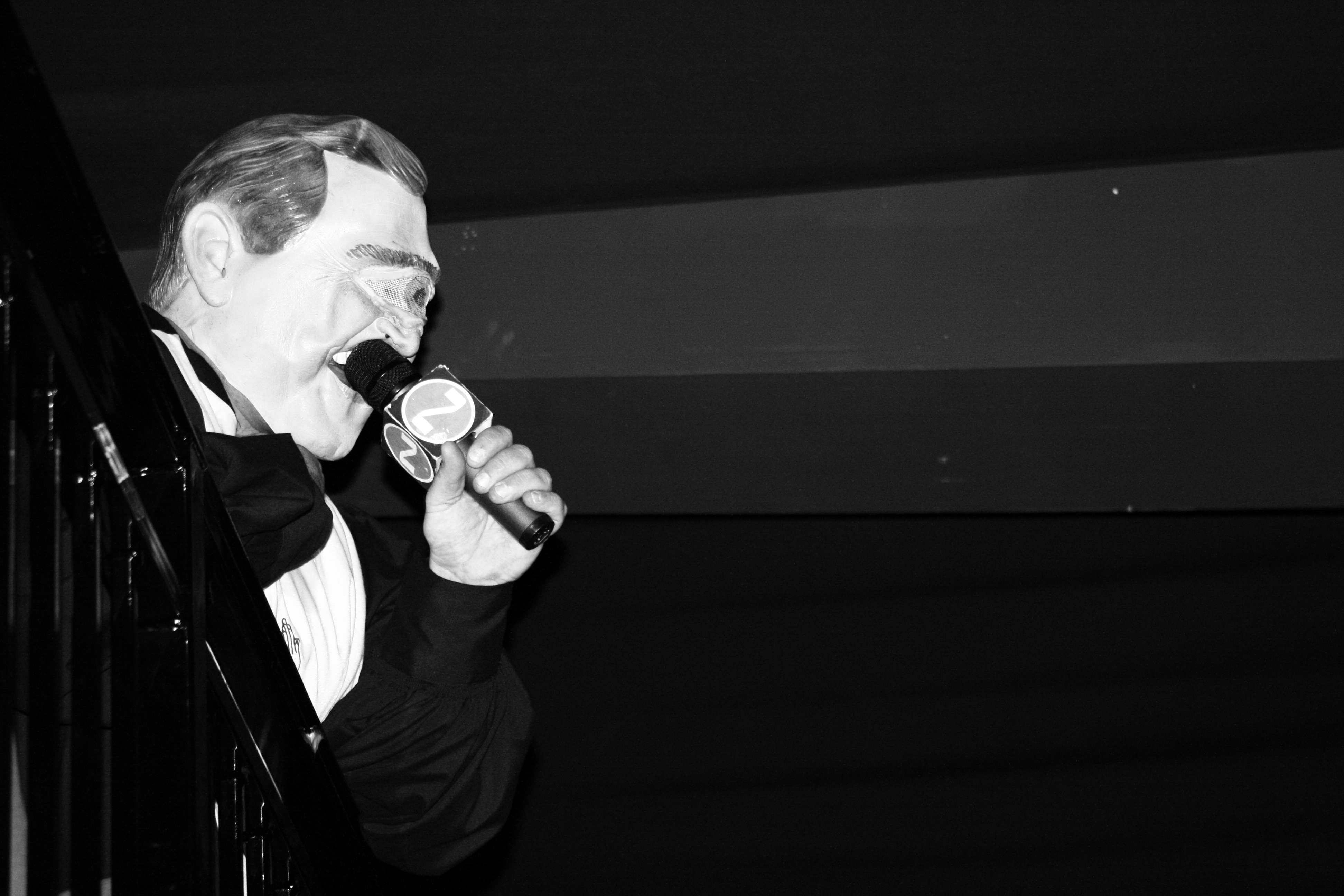### F O T O by F R A N K M O N T E S### F O T O by F R A N K M O N T E S### F O T O by F R A N K M O N T E S### F O T O by F R A N K M O N T E S### F O T O by F R A N K M O N T E S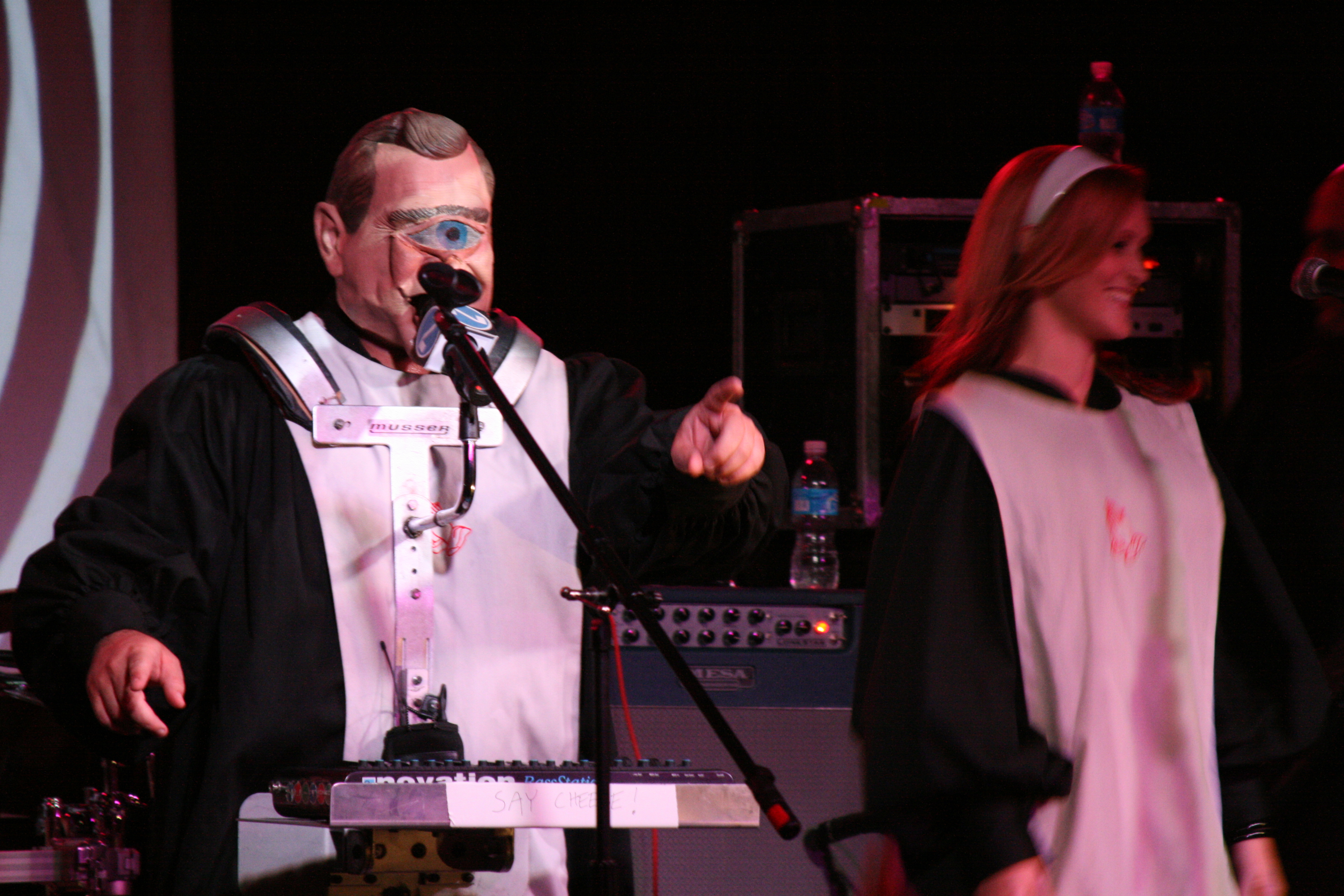### F O T O by F R A N K M O N T E S### F O T O by F R A N K M O N T E S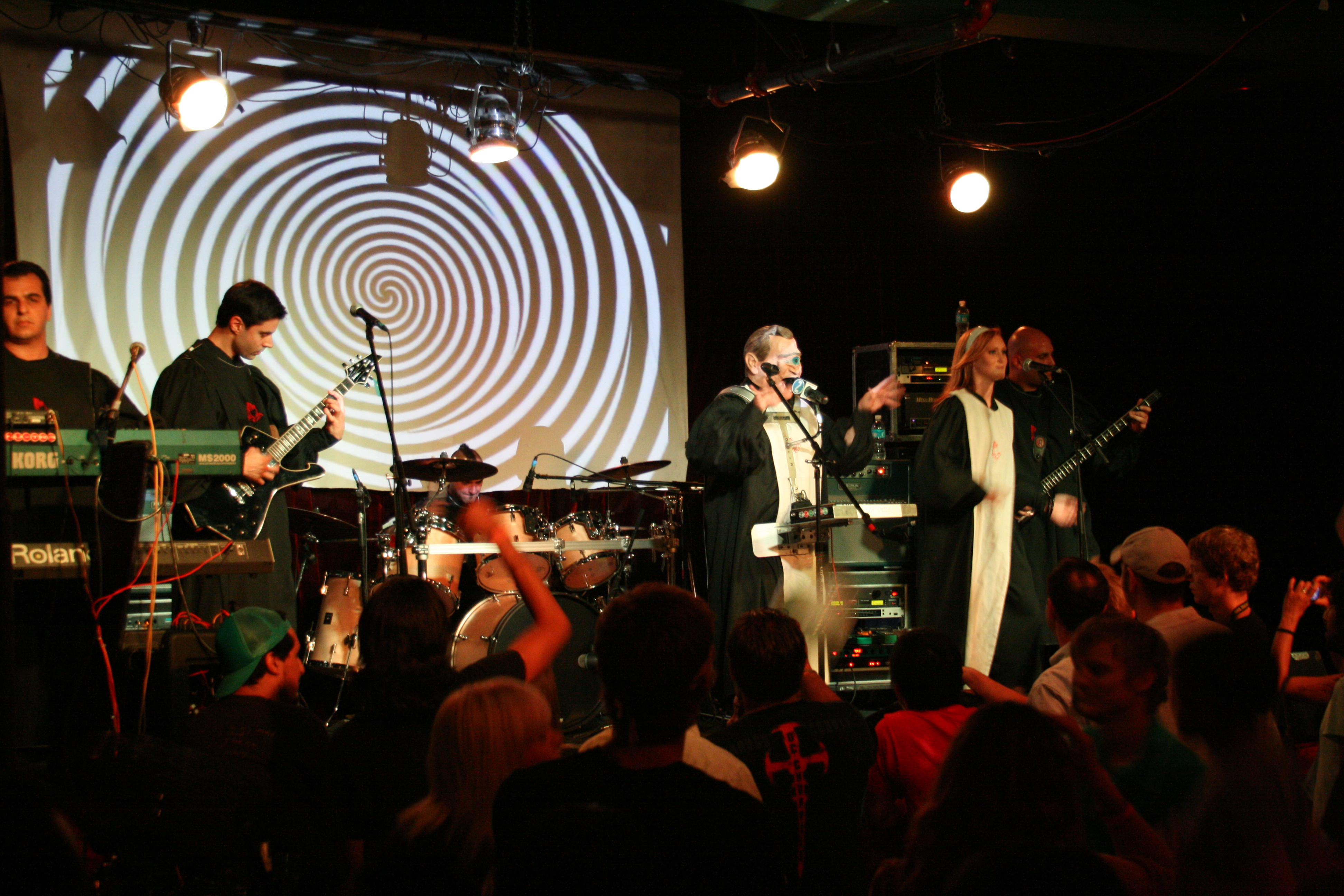### F O T O by F R A N K M O N T E S